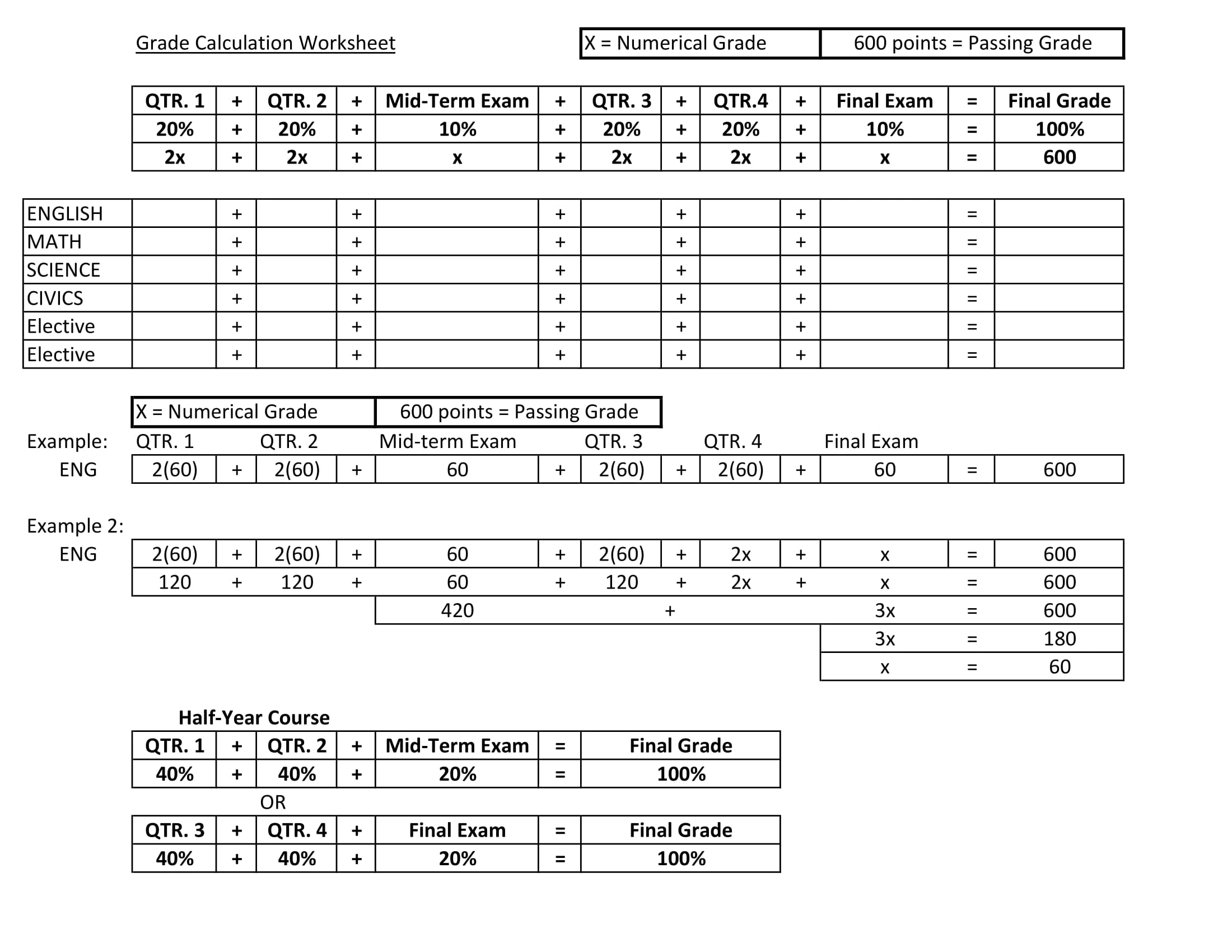# Worksheets and Assignments

Your resource for free worksheets and practice assignments covering topics ranging from graphing calculators to mathematical, scientific, and statistical problems. These printable worksheets can be downloaded for free for students, teachers, parents, and homeschoolers. Work on these practice tests conveniently with our free comprehensive online calculators available here.

Graphing Calculator Basics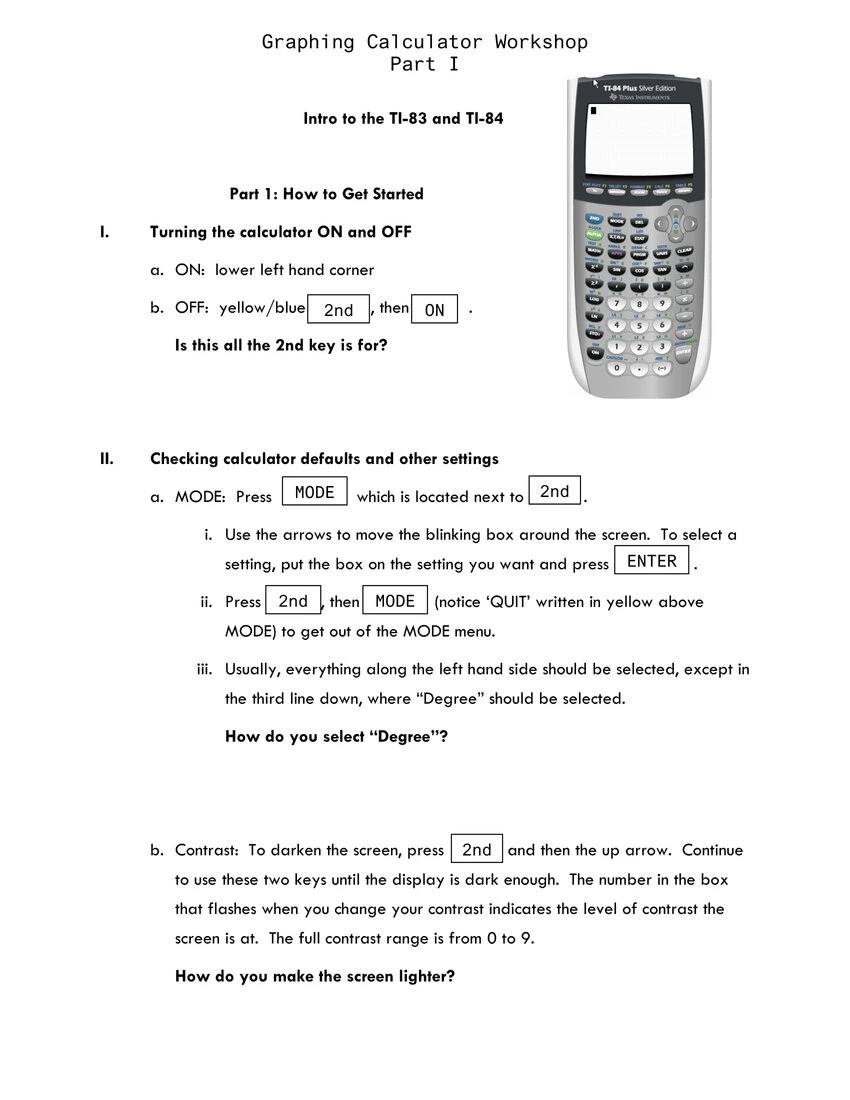Exponential & Logarithm Handout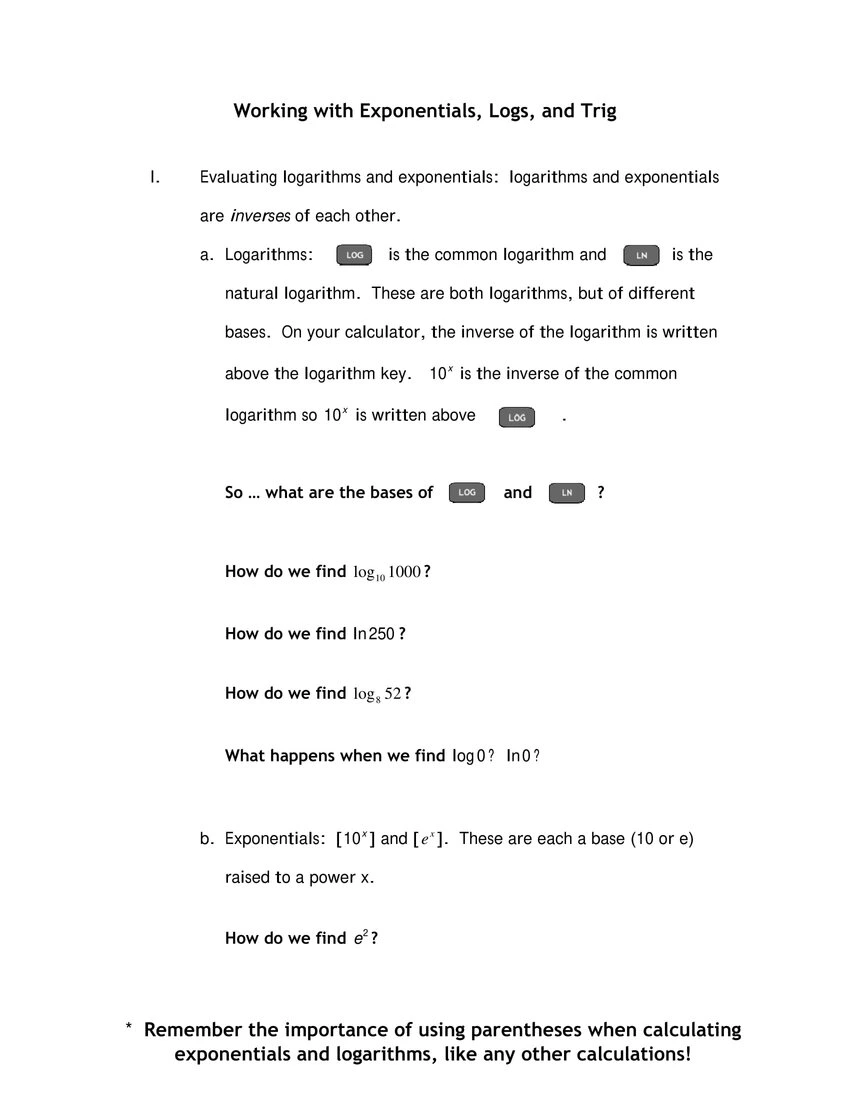Trigonometric & Polar GraphsHow to Graph Linear Functions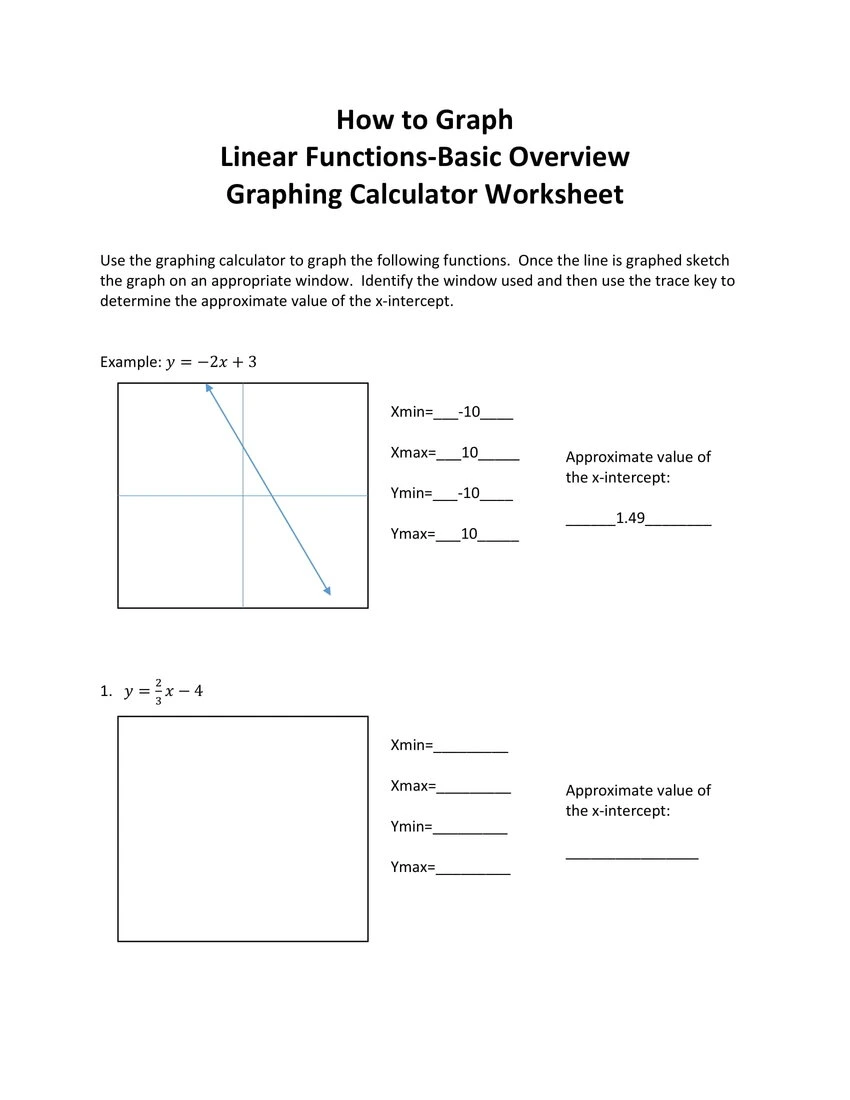Scatter Plots with a Graphing Calculator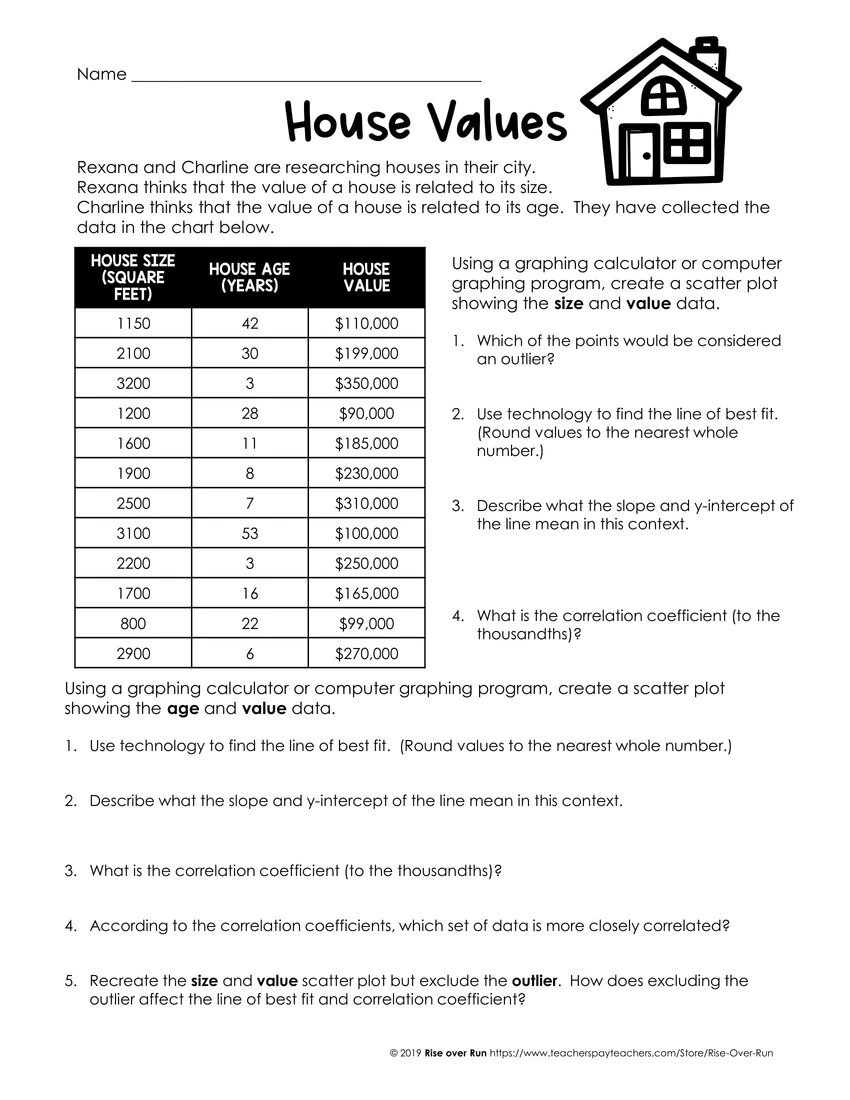Features of Quadratic Functions Worksheet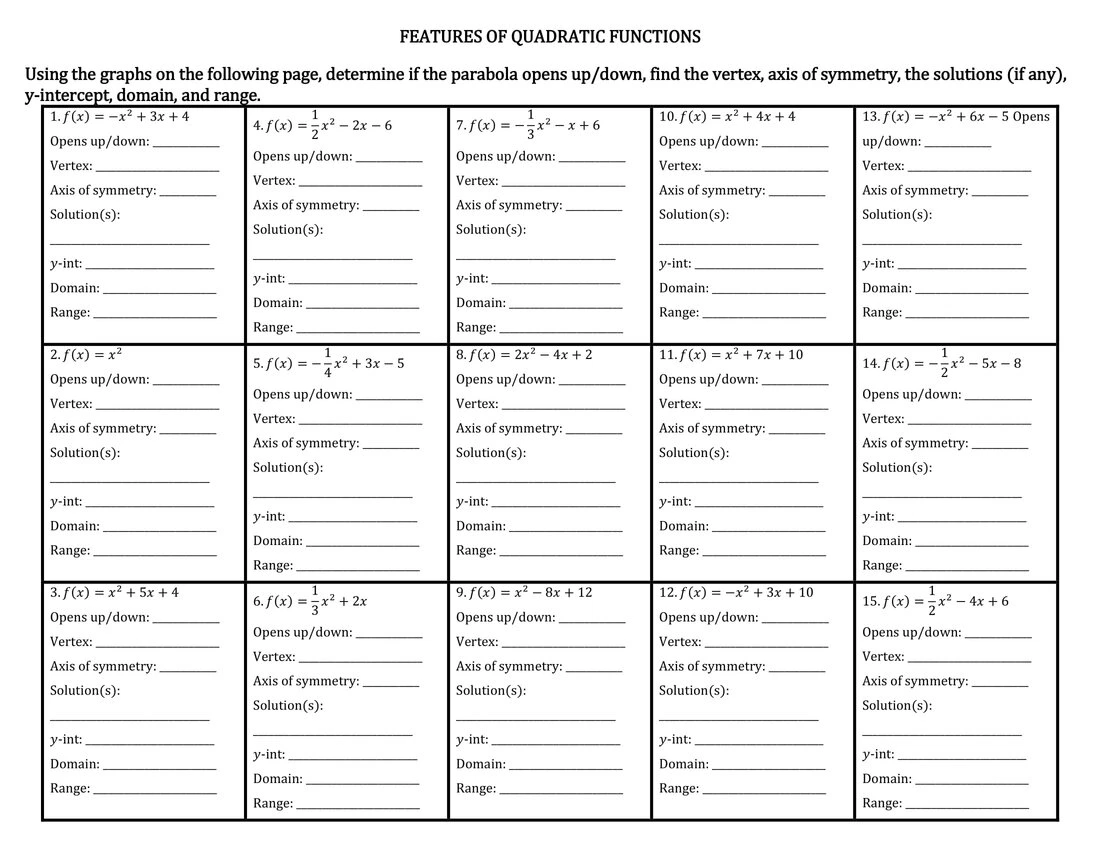Investigating Shifts of Absolute Value Graphs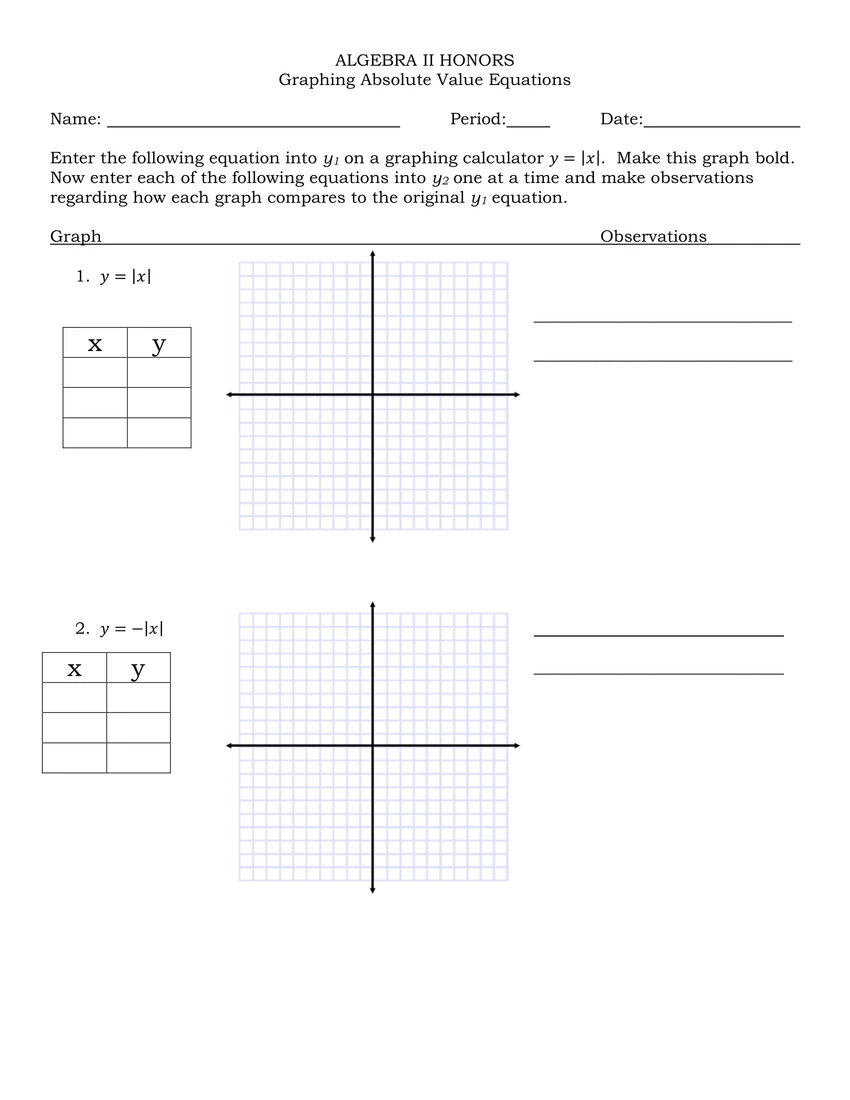Exploring Rational FunctionsStandard Deviation ActivitiesData Summary, Presentation, & Normal DistributionStandard Deviation Practice WorksheetVariance and Standard DeviationFinding the Standard Deviation with Population VarianceHalloween-Themed Statistics Activity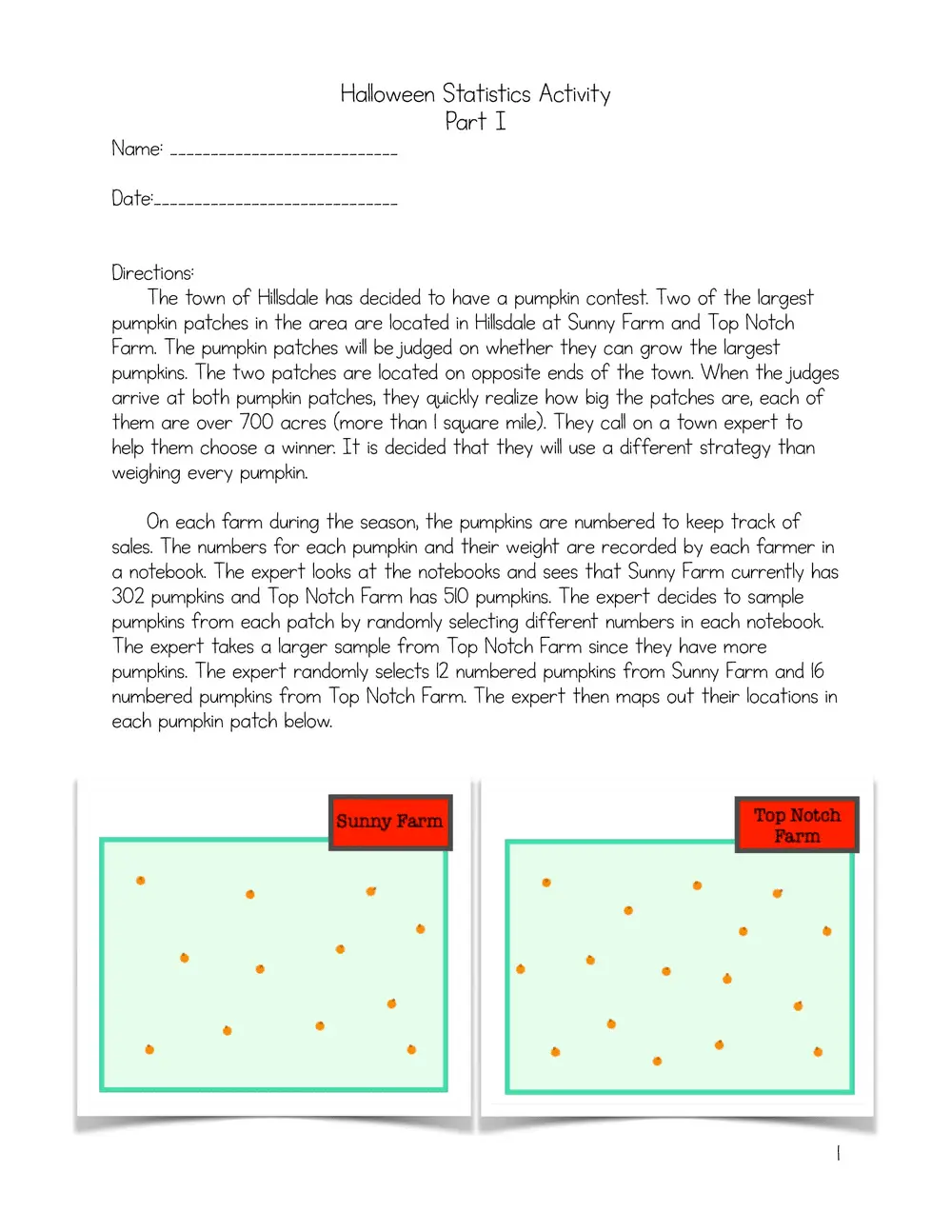Dice Activity for Standard Deviation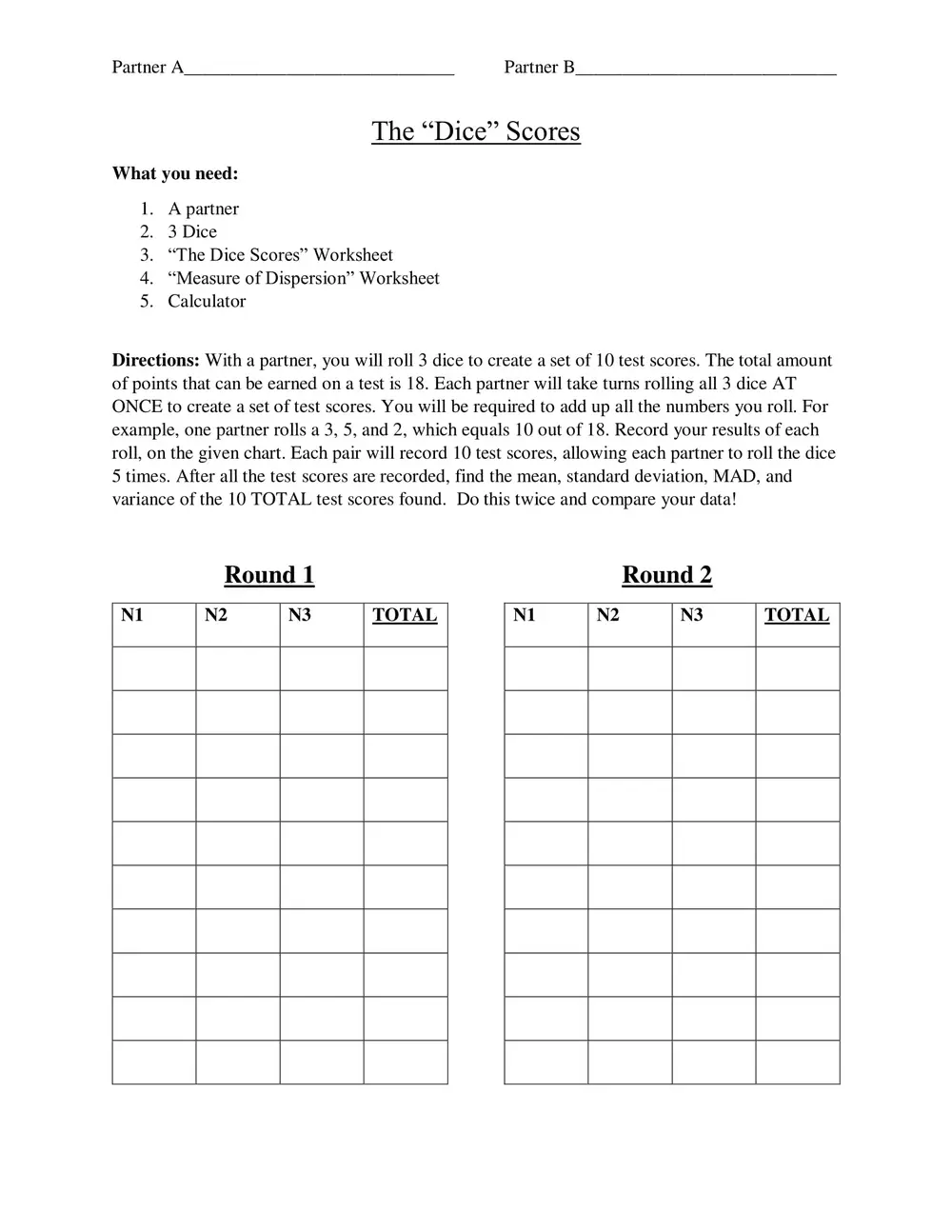Lysozyme Experiment Analysis: Calculating Standard Deviation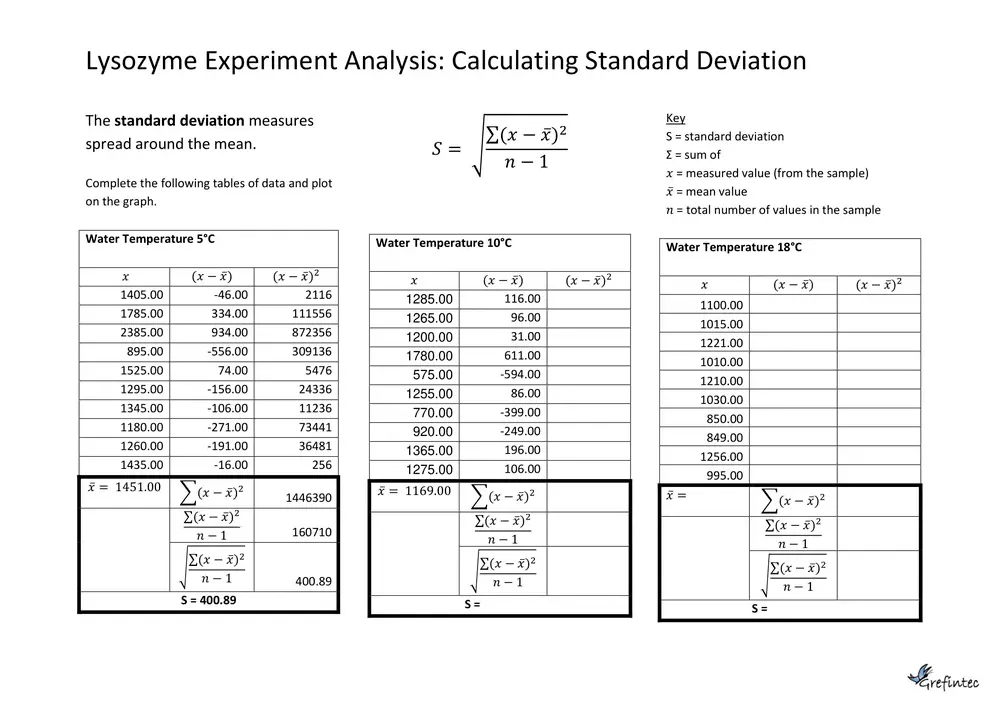Estimating π – scientific calculator practice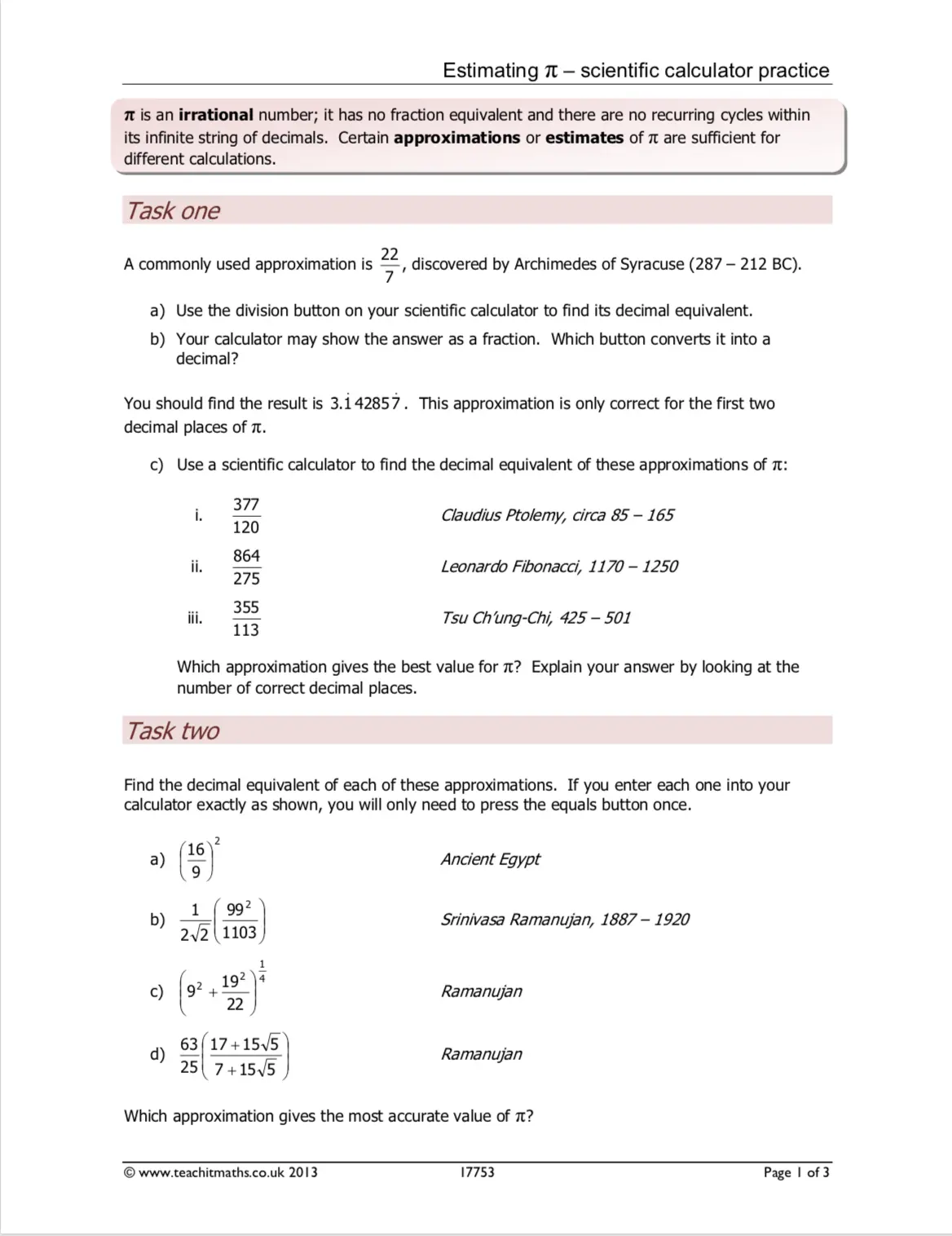Logarithm & Exponent Review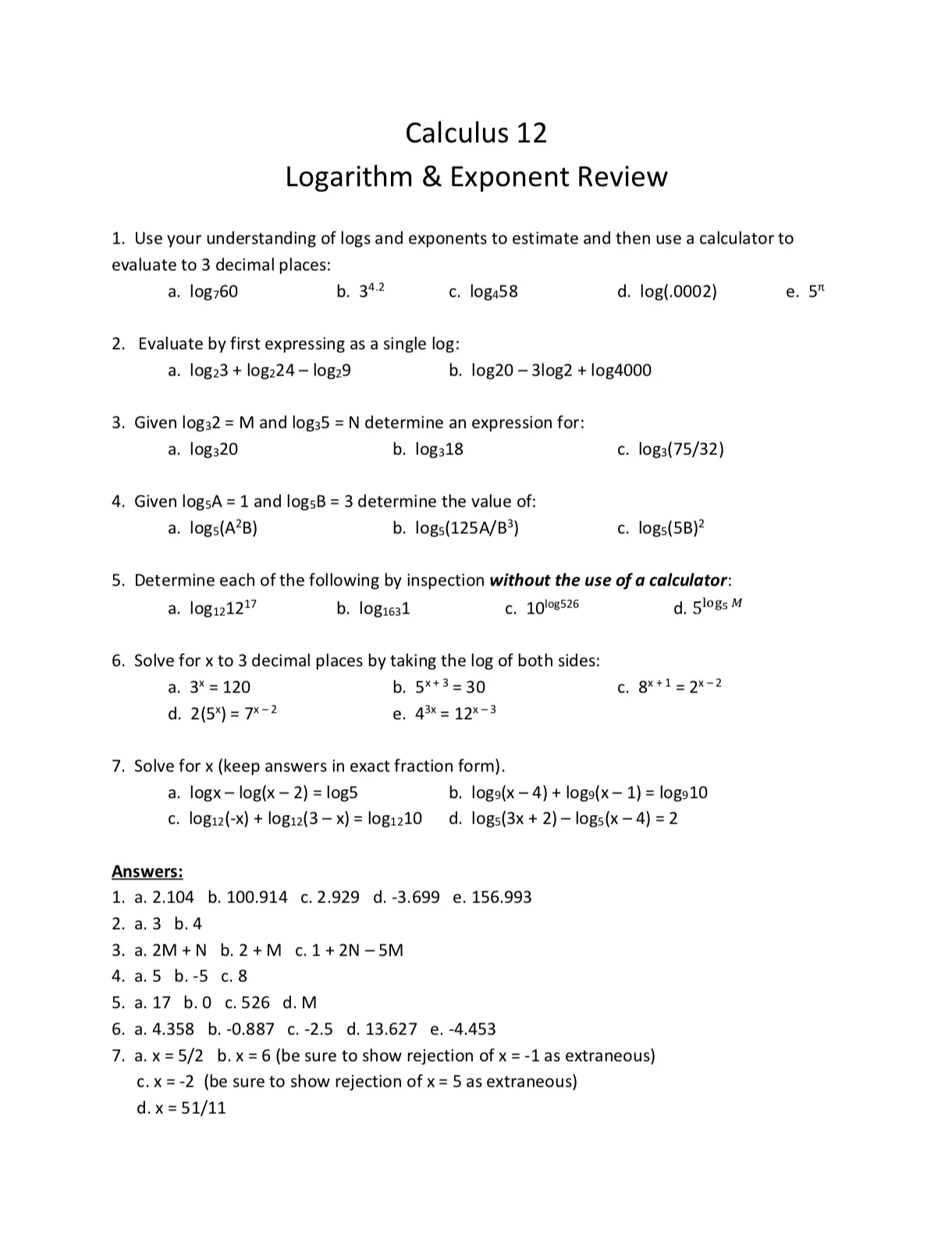Index & Logarithmic Notation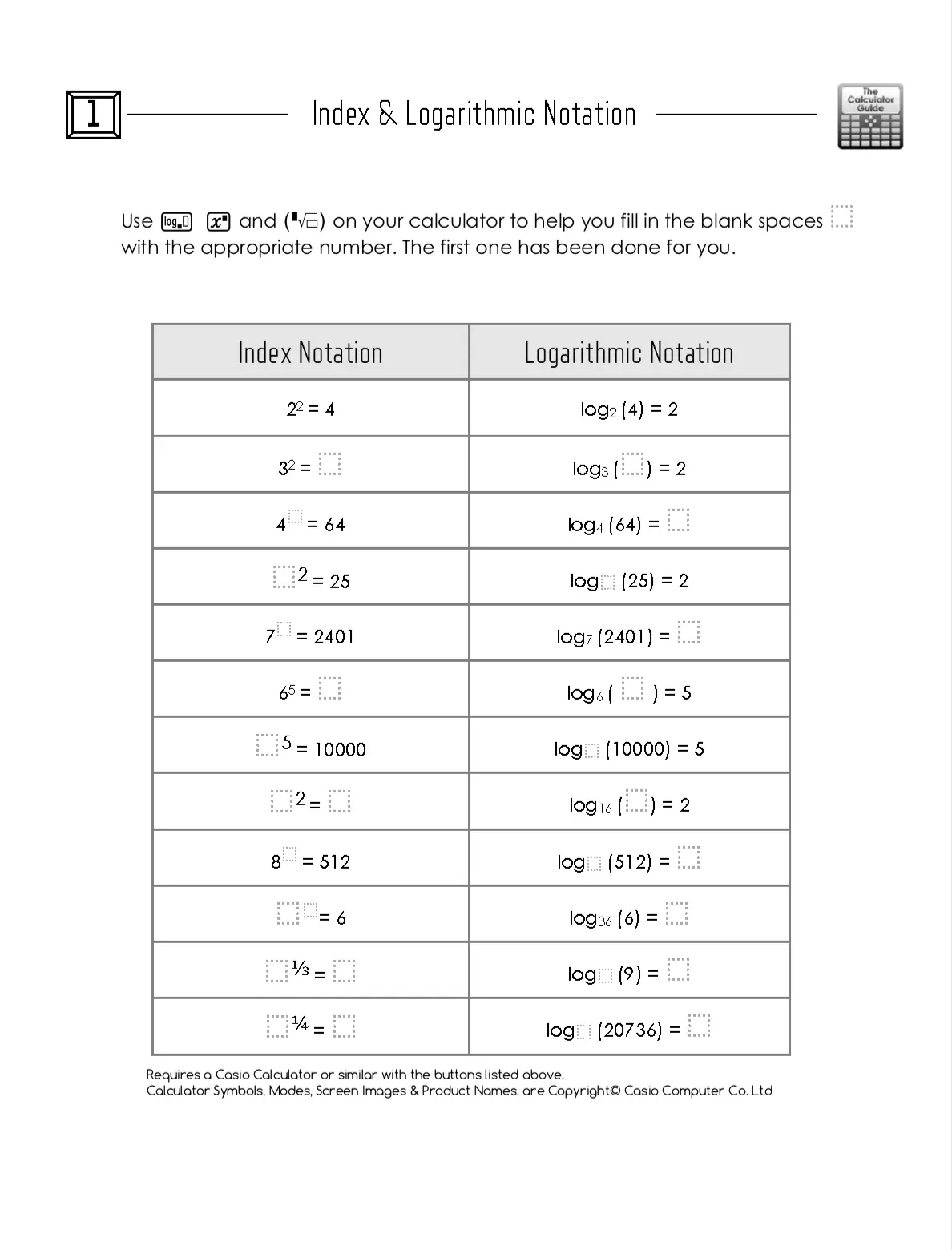Introduction to Trigonometry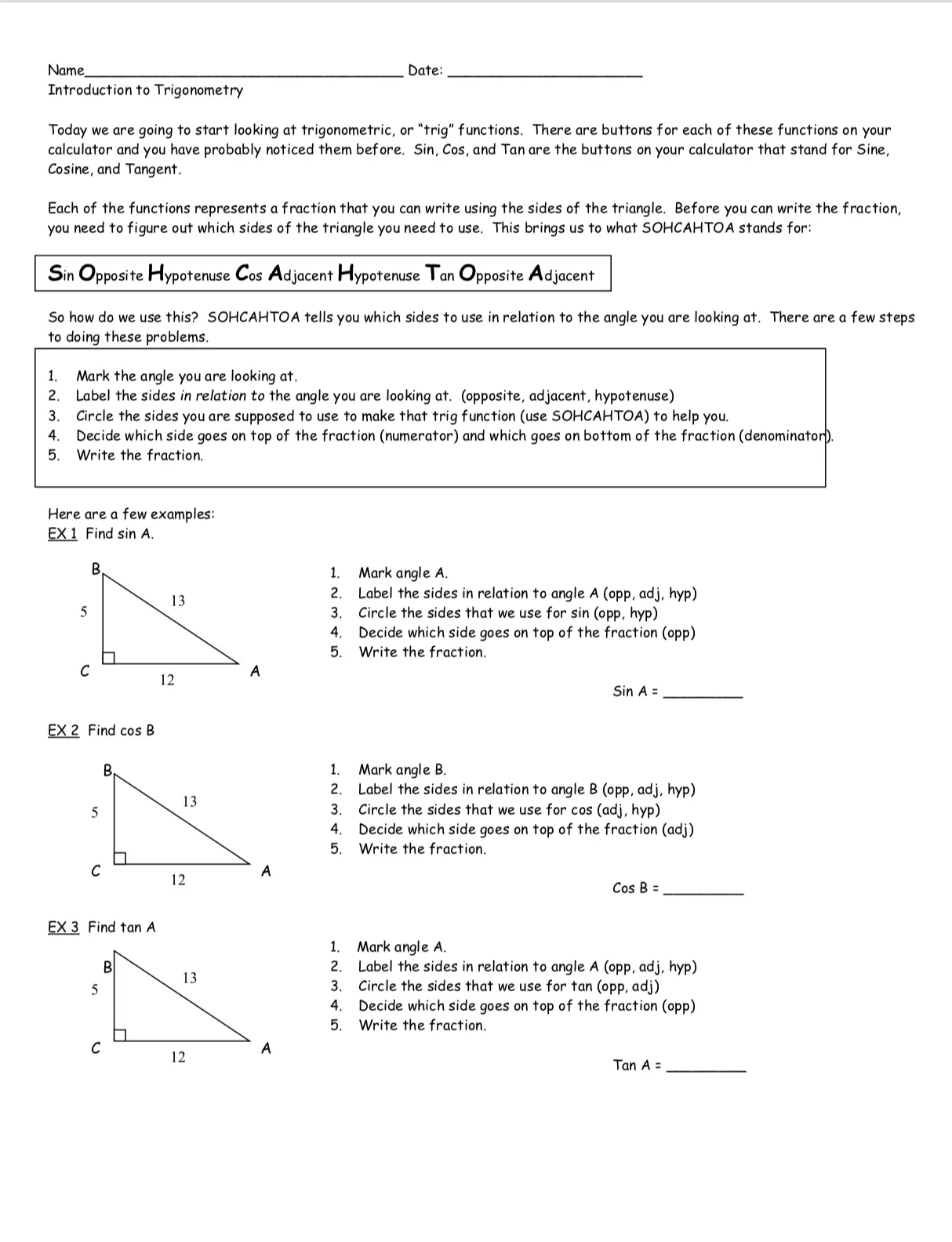Multiplying and Dividing Using Scientific Notation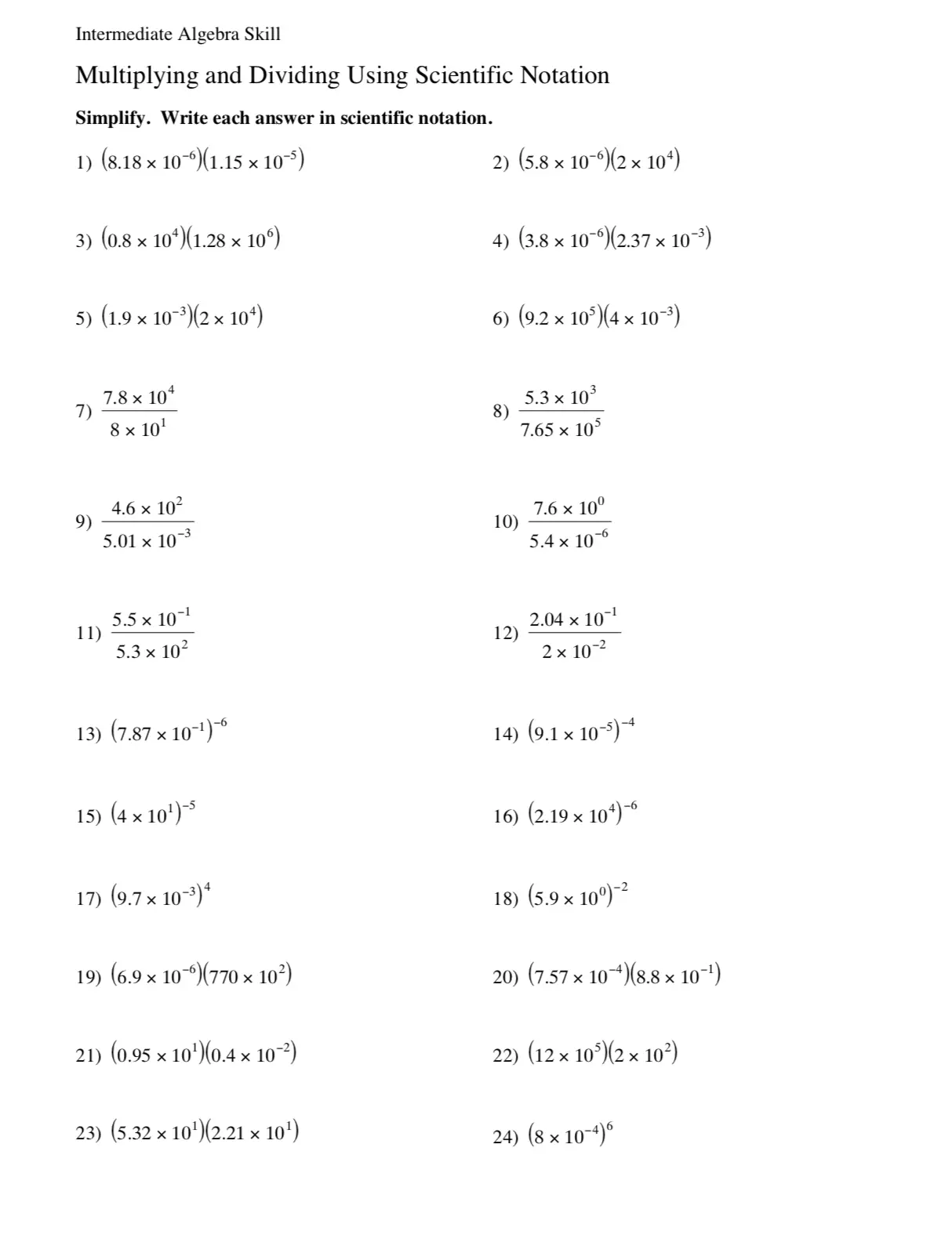Conversions and Scientific Notation Prefixes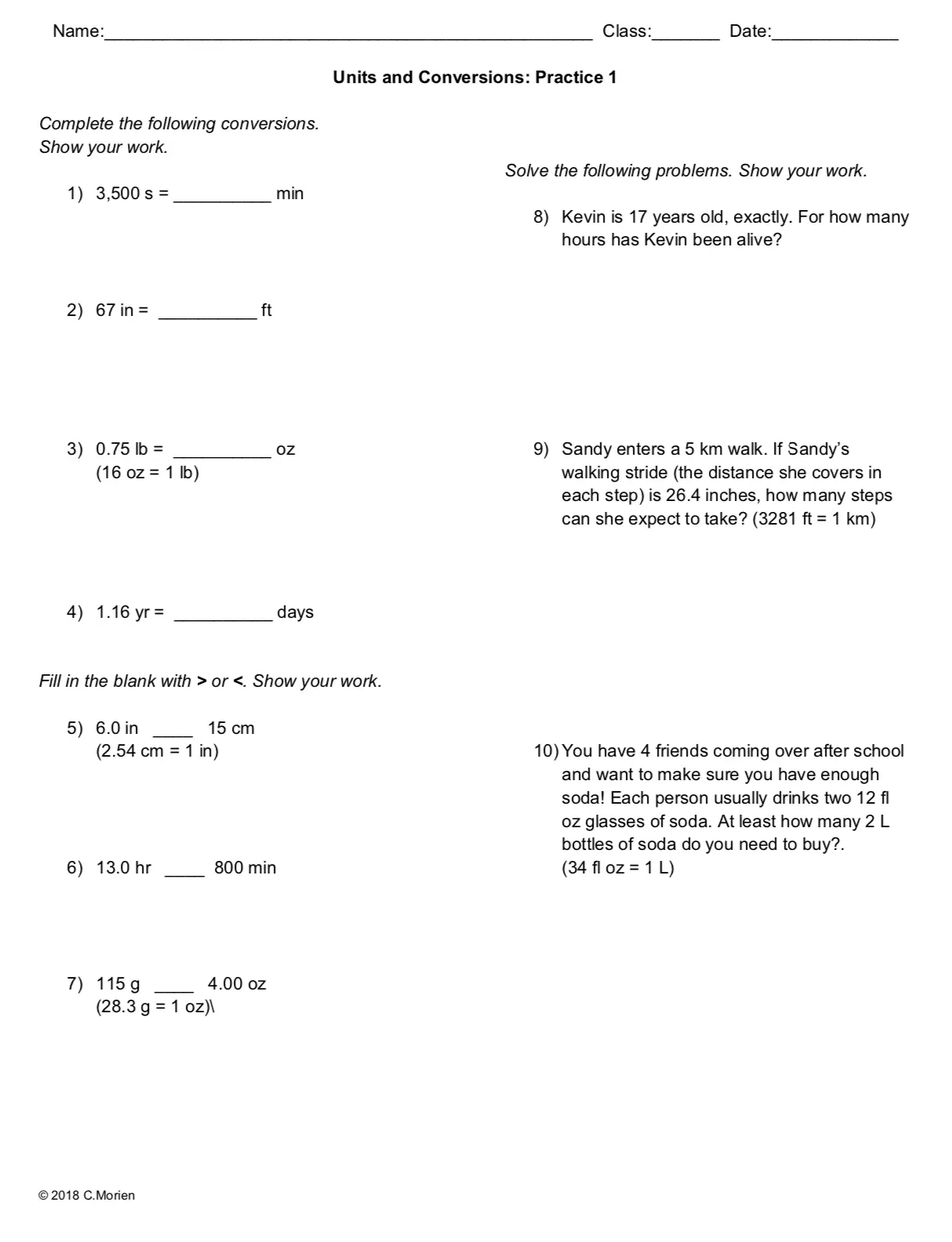Permutations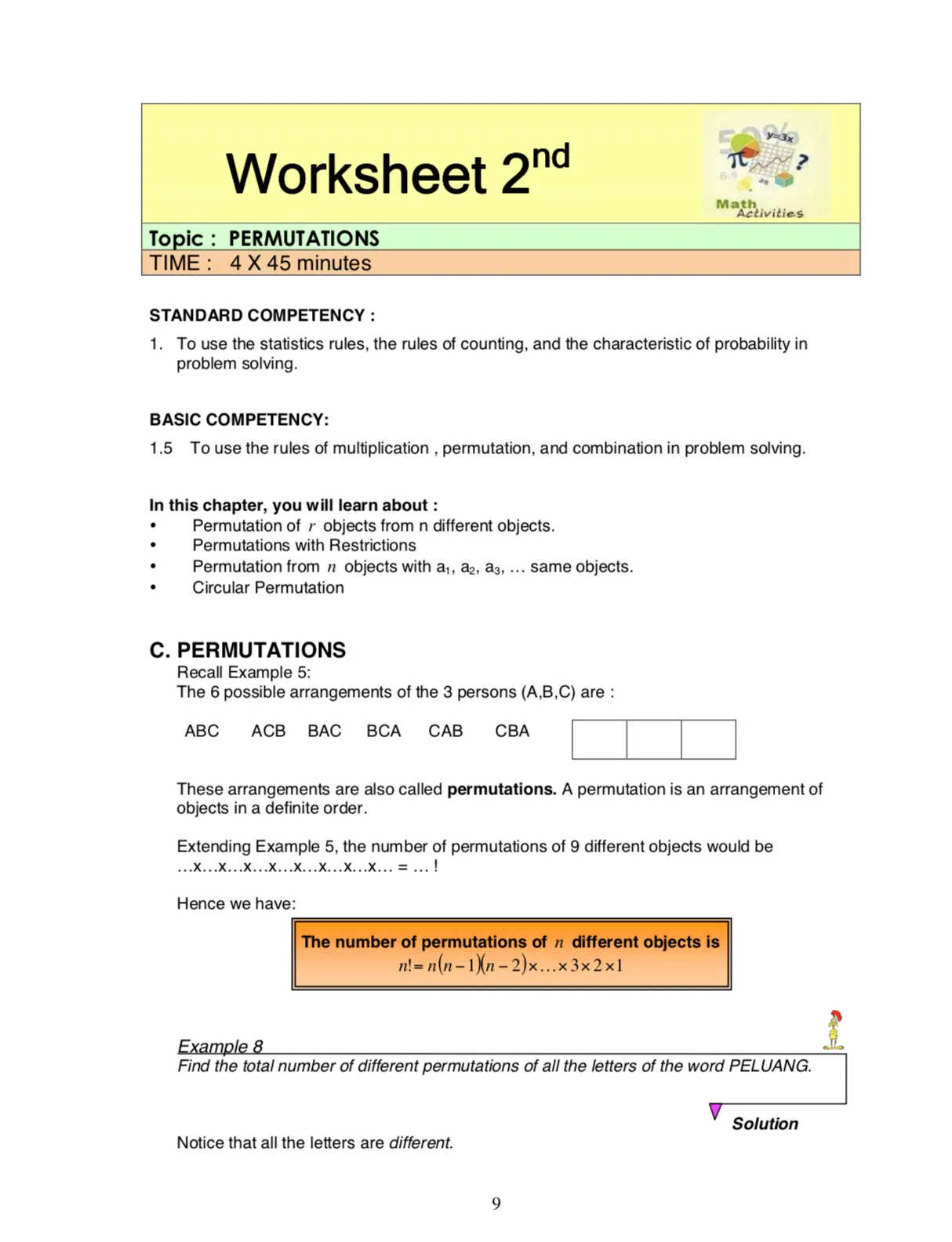Permutations and Combinations Worksheet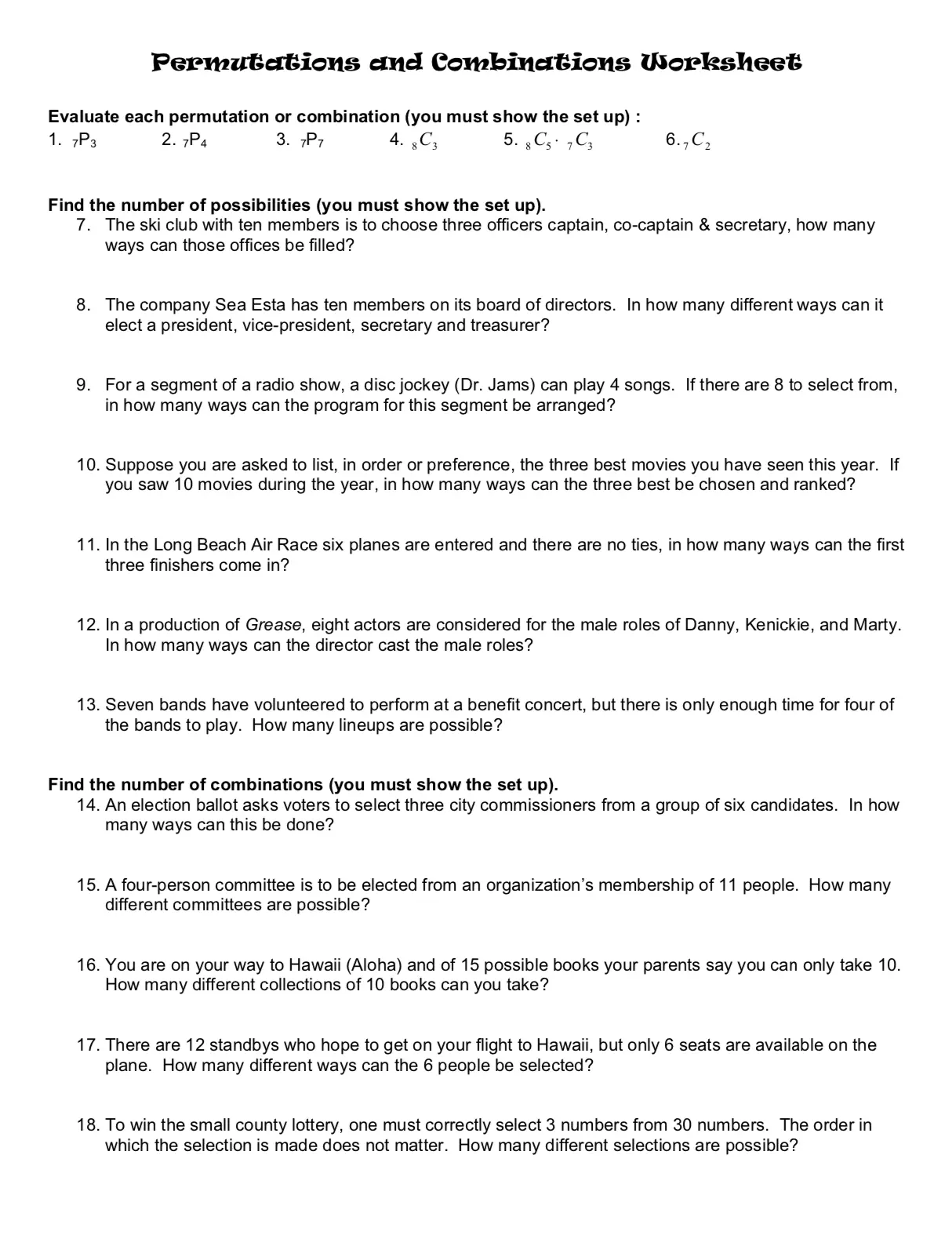Statistics Worksheet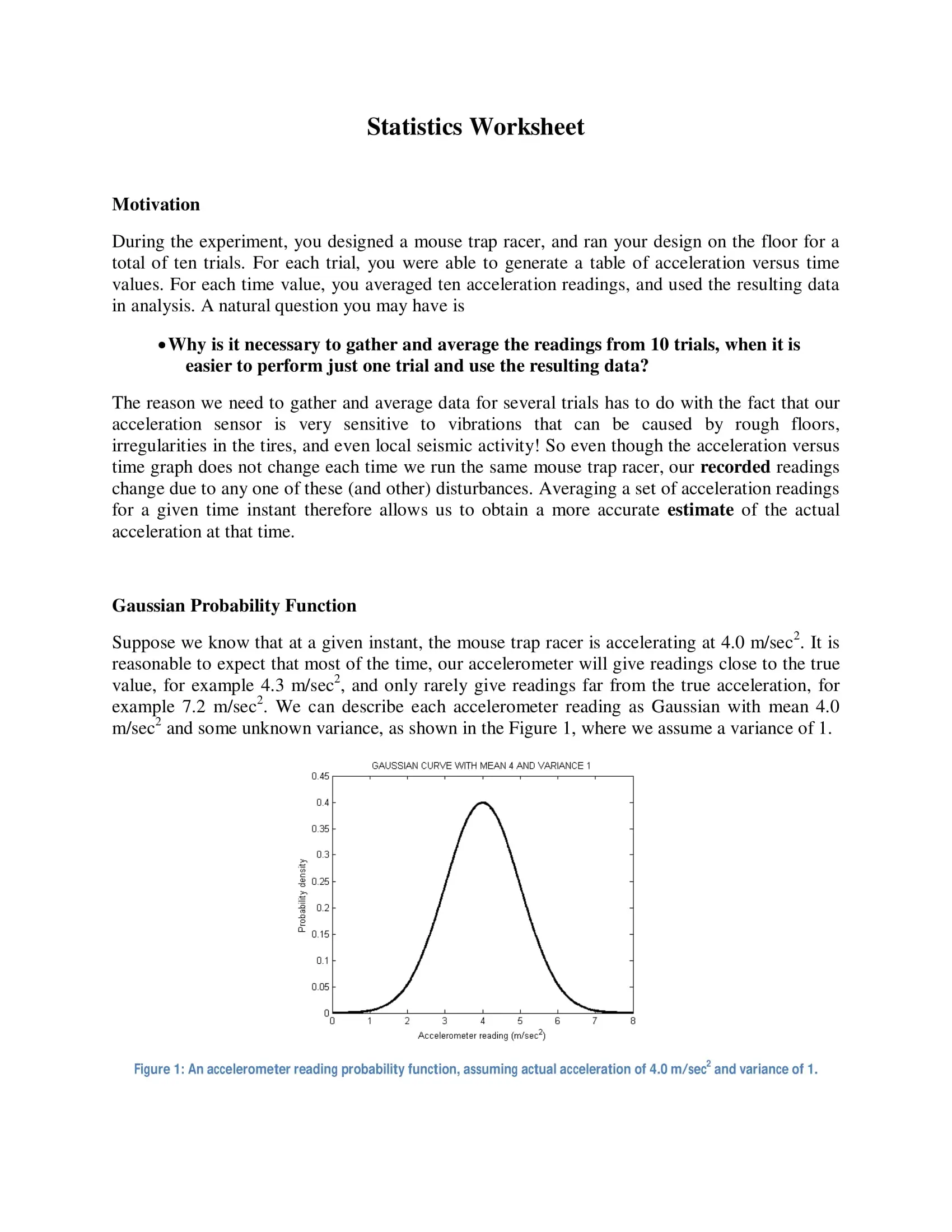Interpreting Linear Data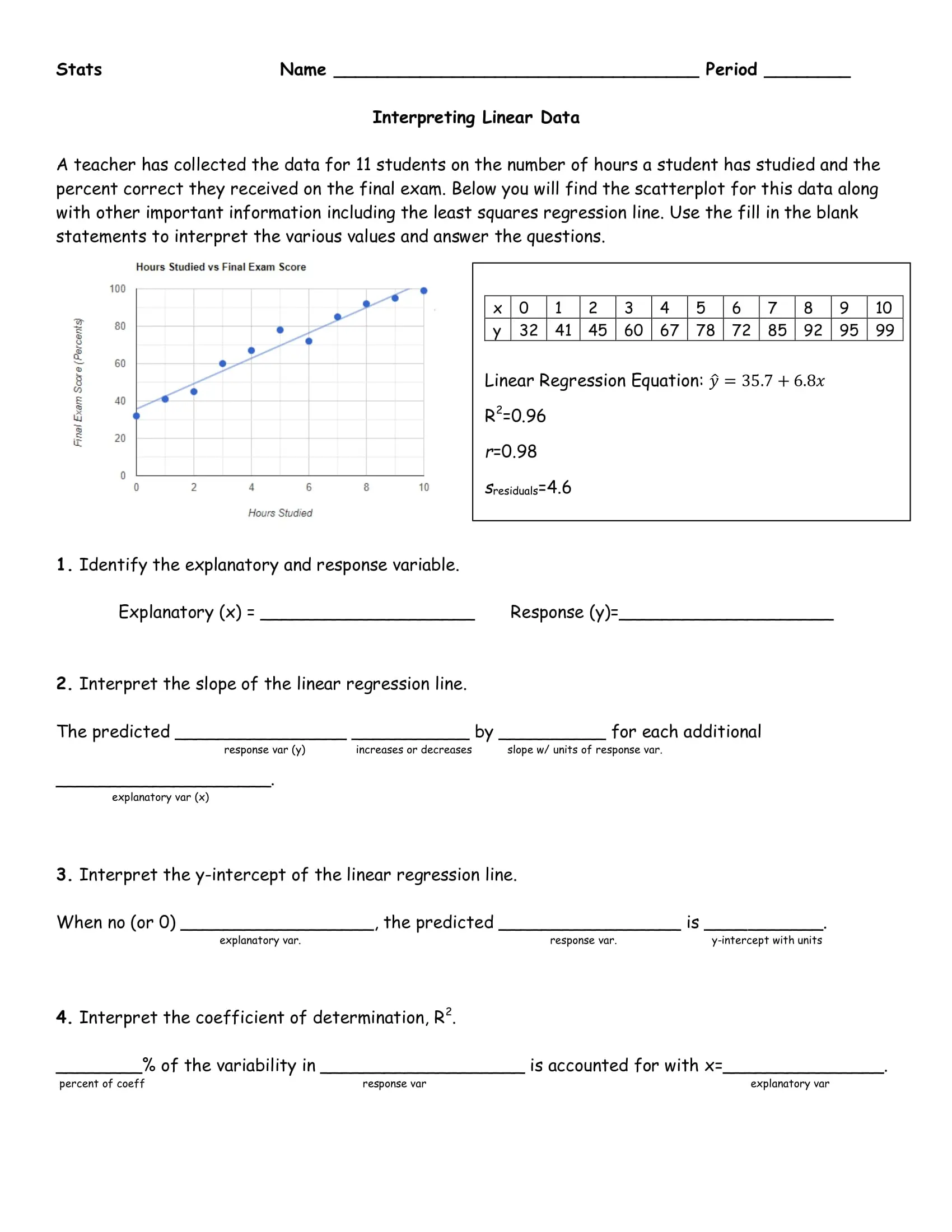Probability Statistics WorksheetStatistics & Fantasy Baseball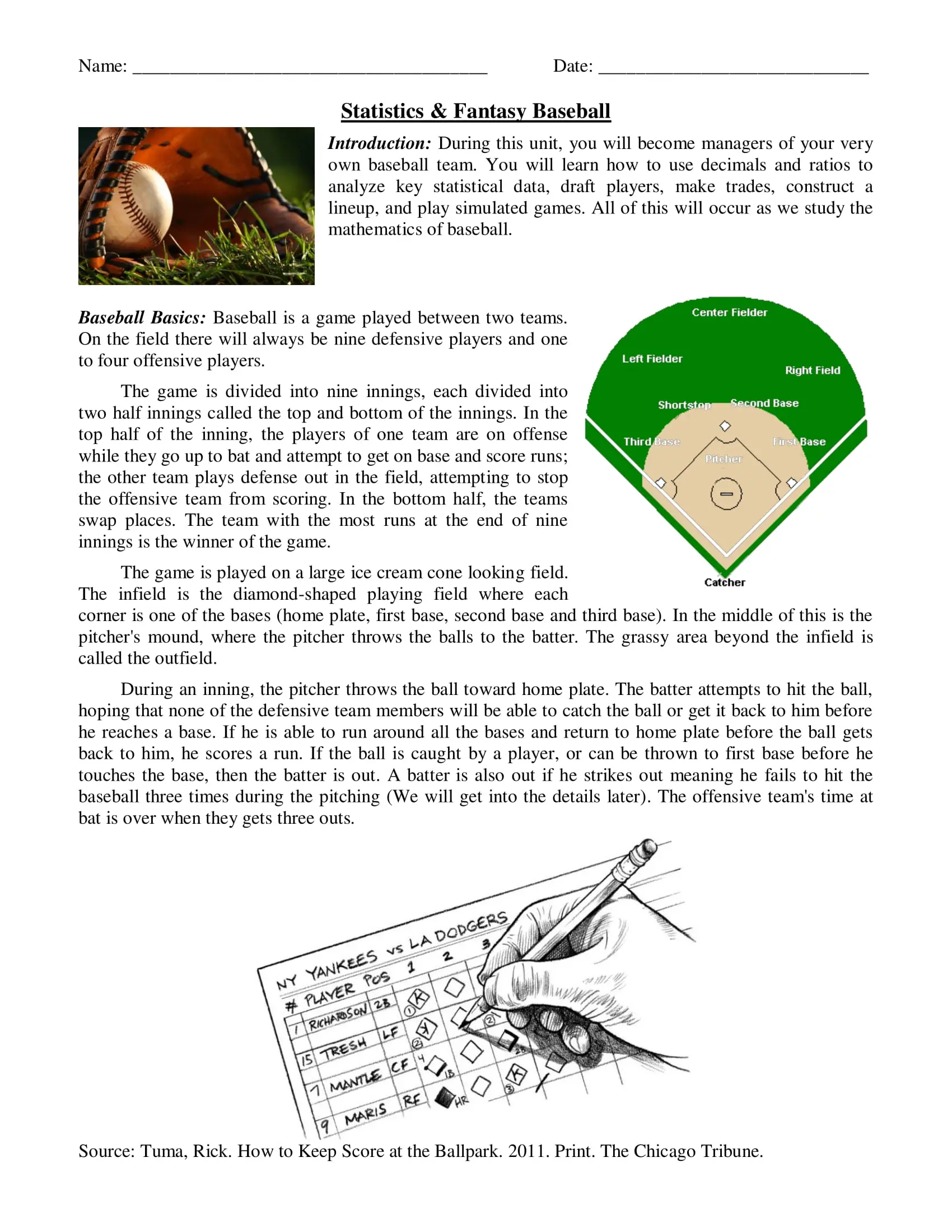Mean, Median, Mode, and Range Project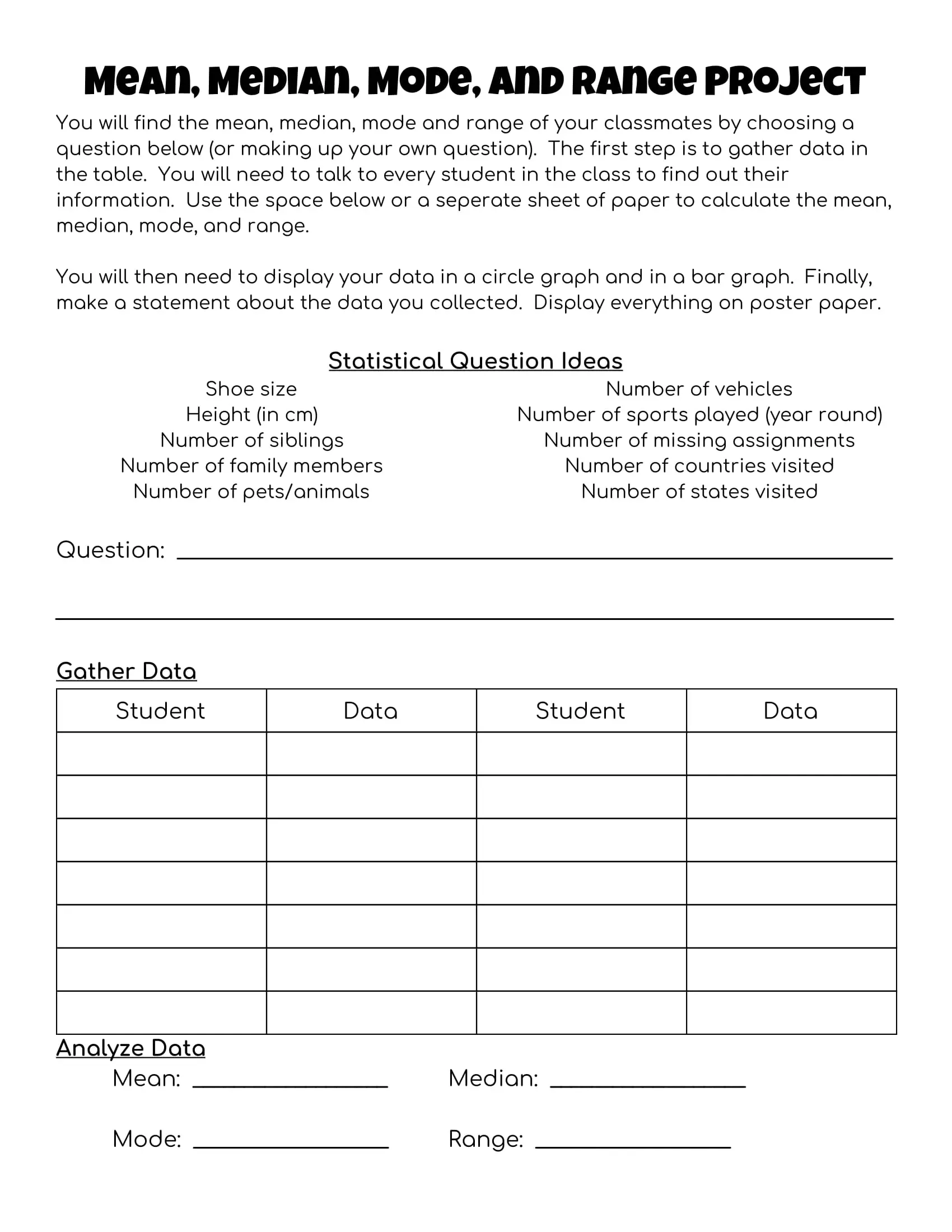Worksheet of Statistics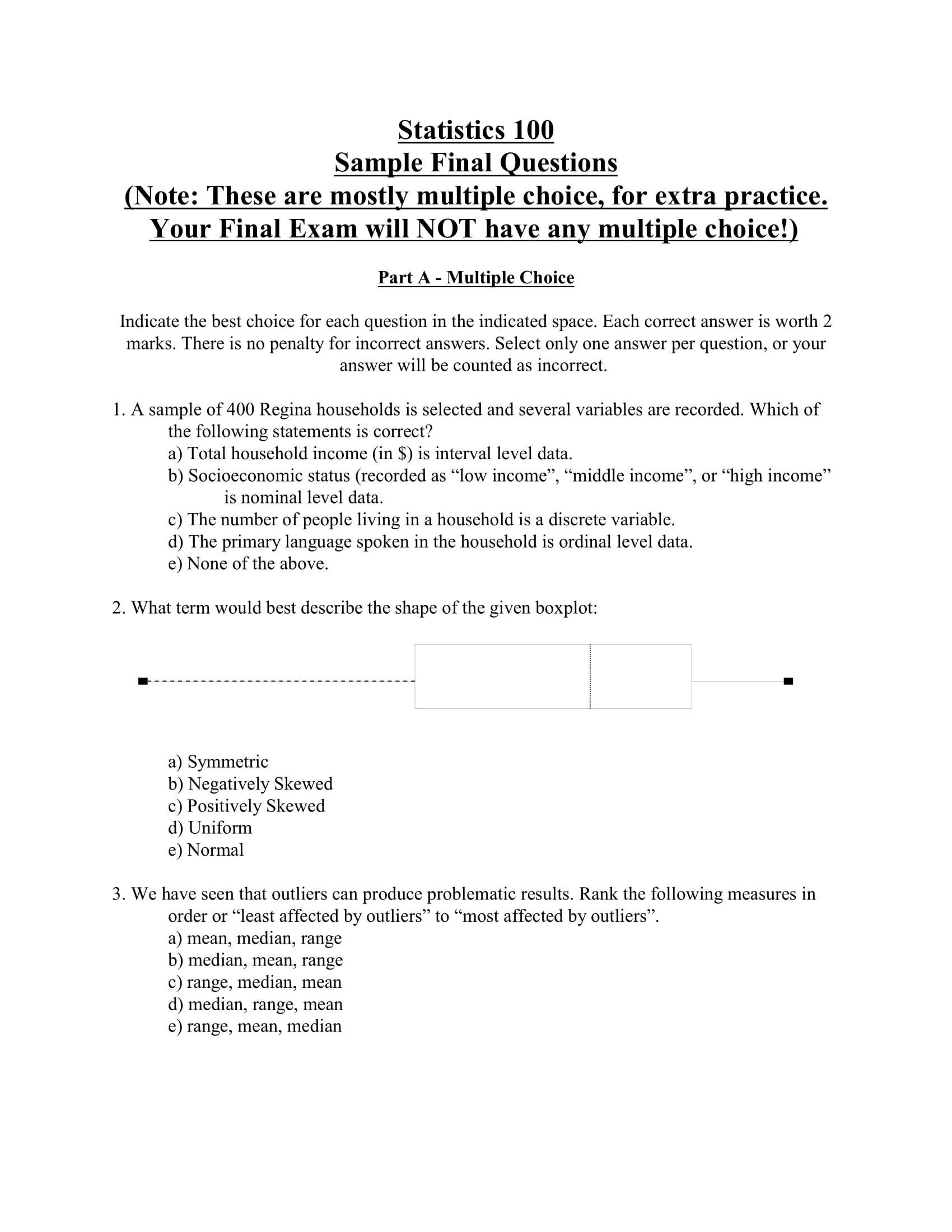Sample Statistics Worksheet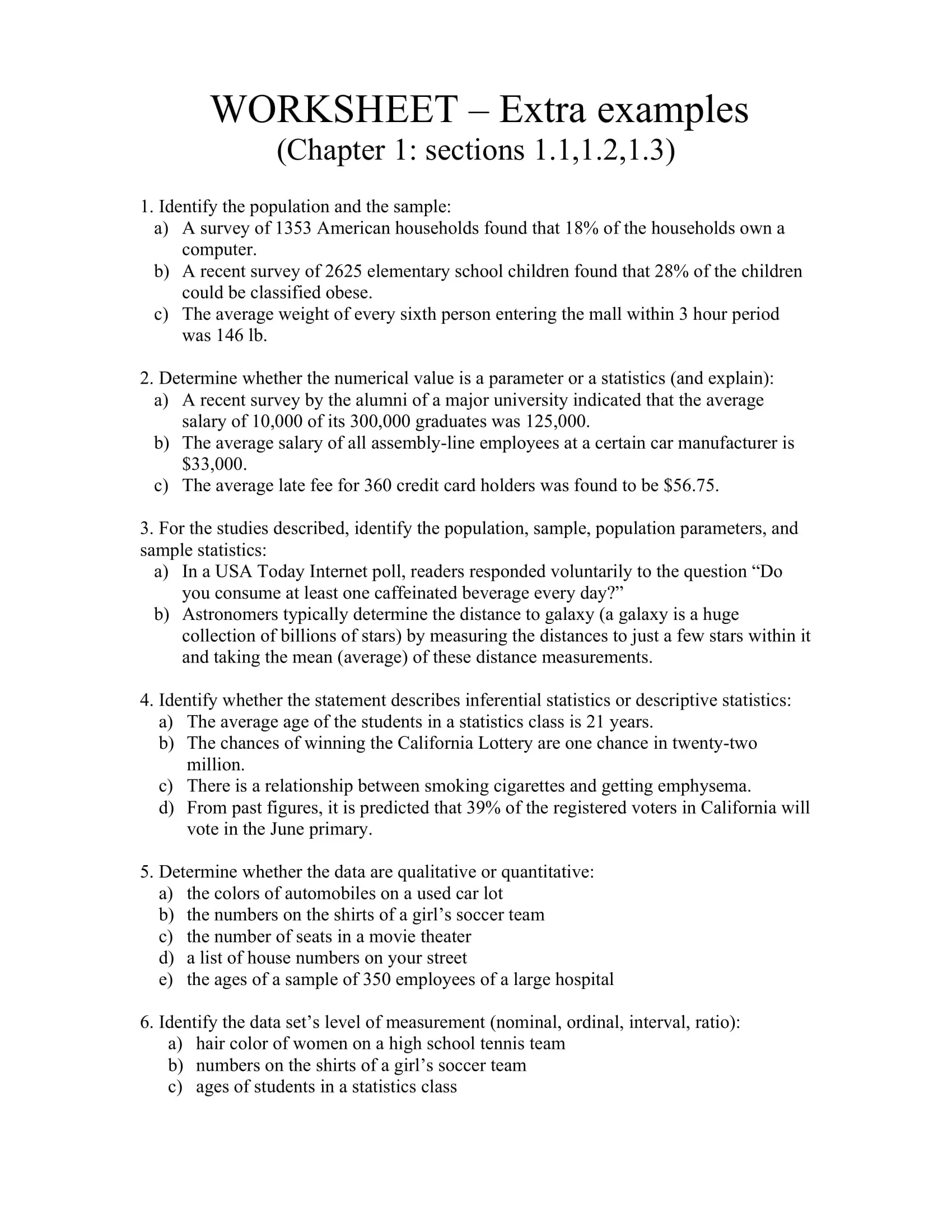Super Bowl Math - Statistics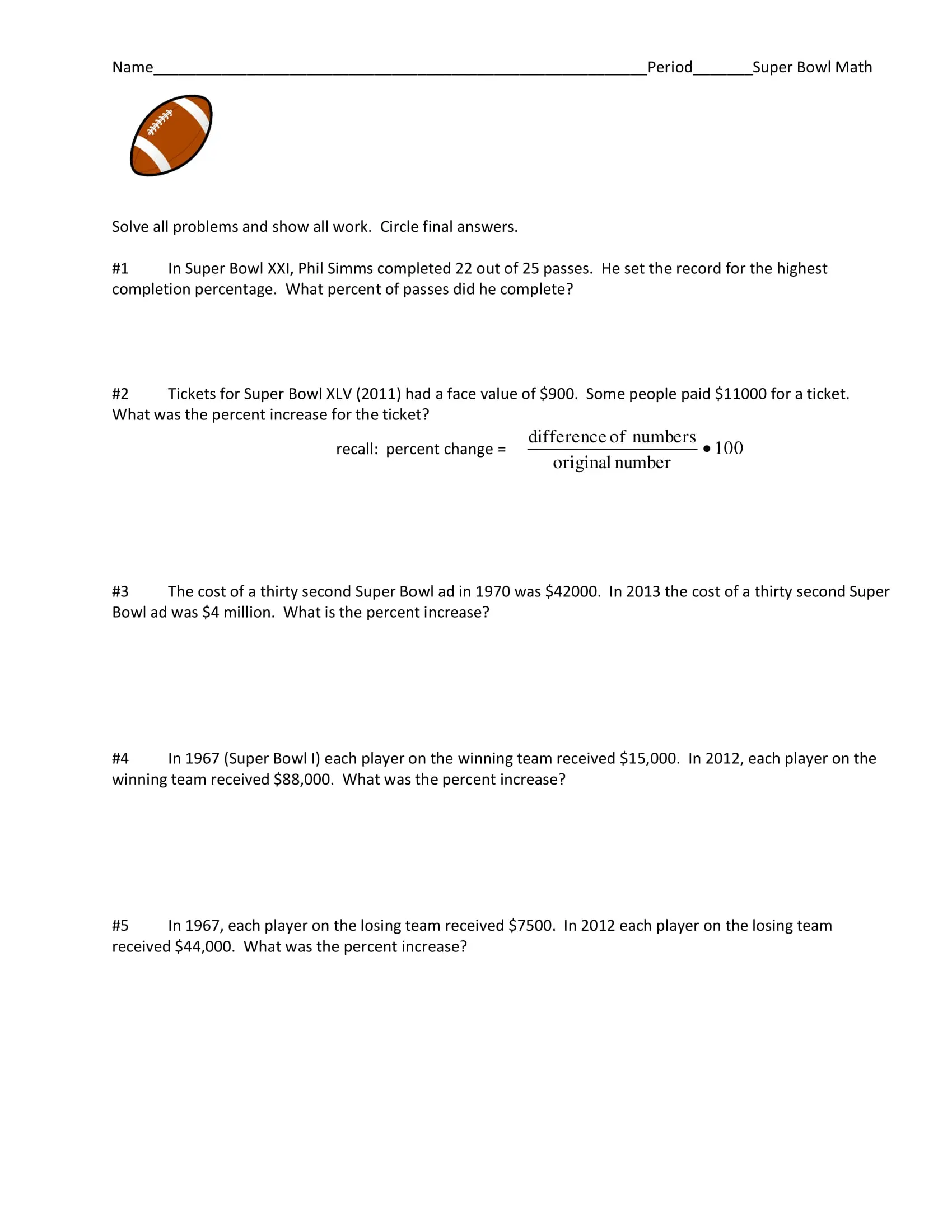Unpaired and Paired t-Test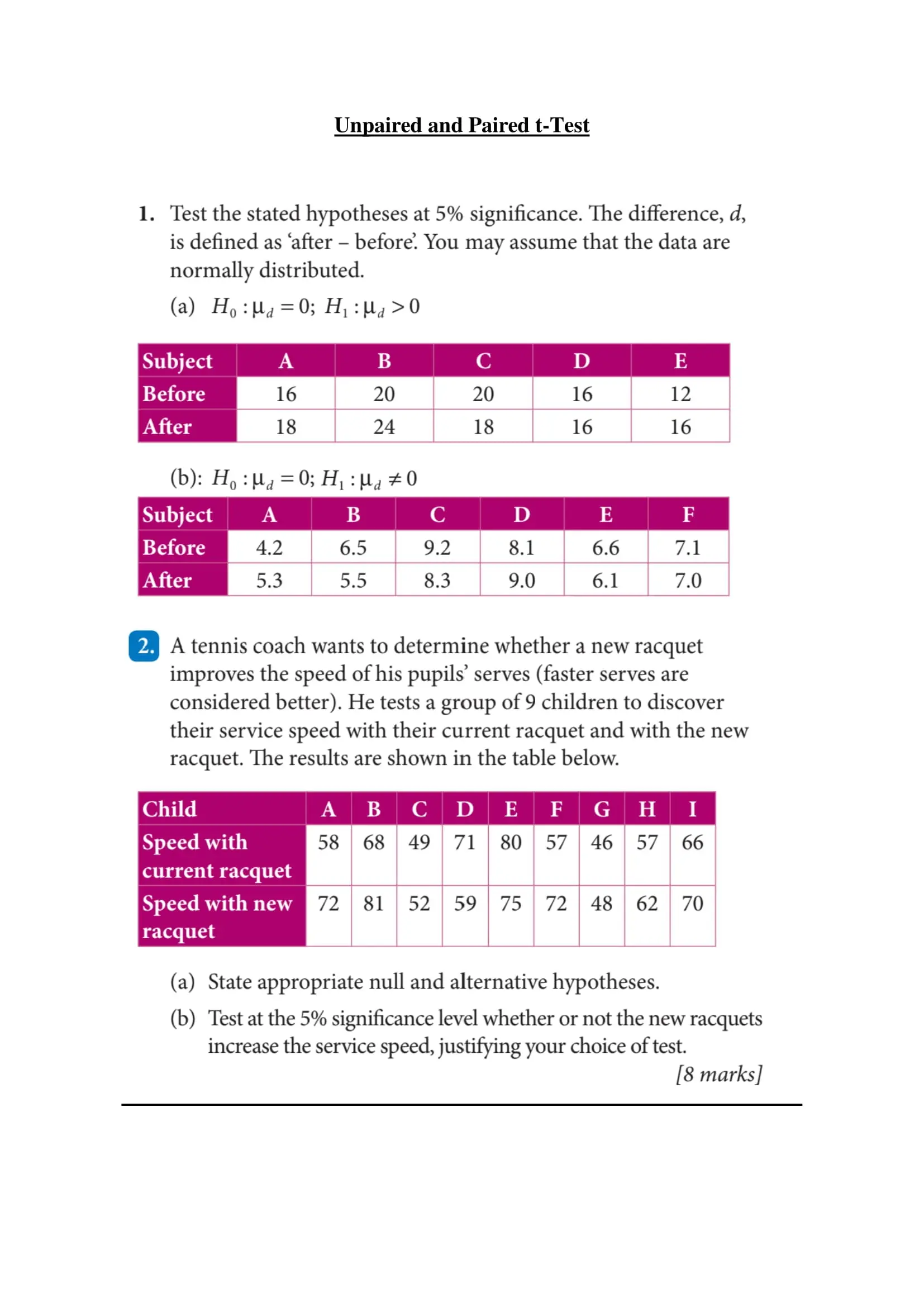T-Test Worksheet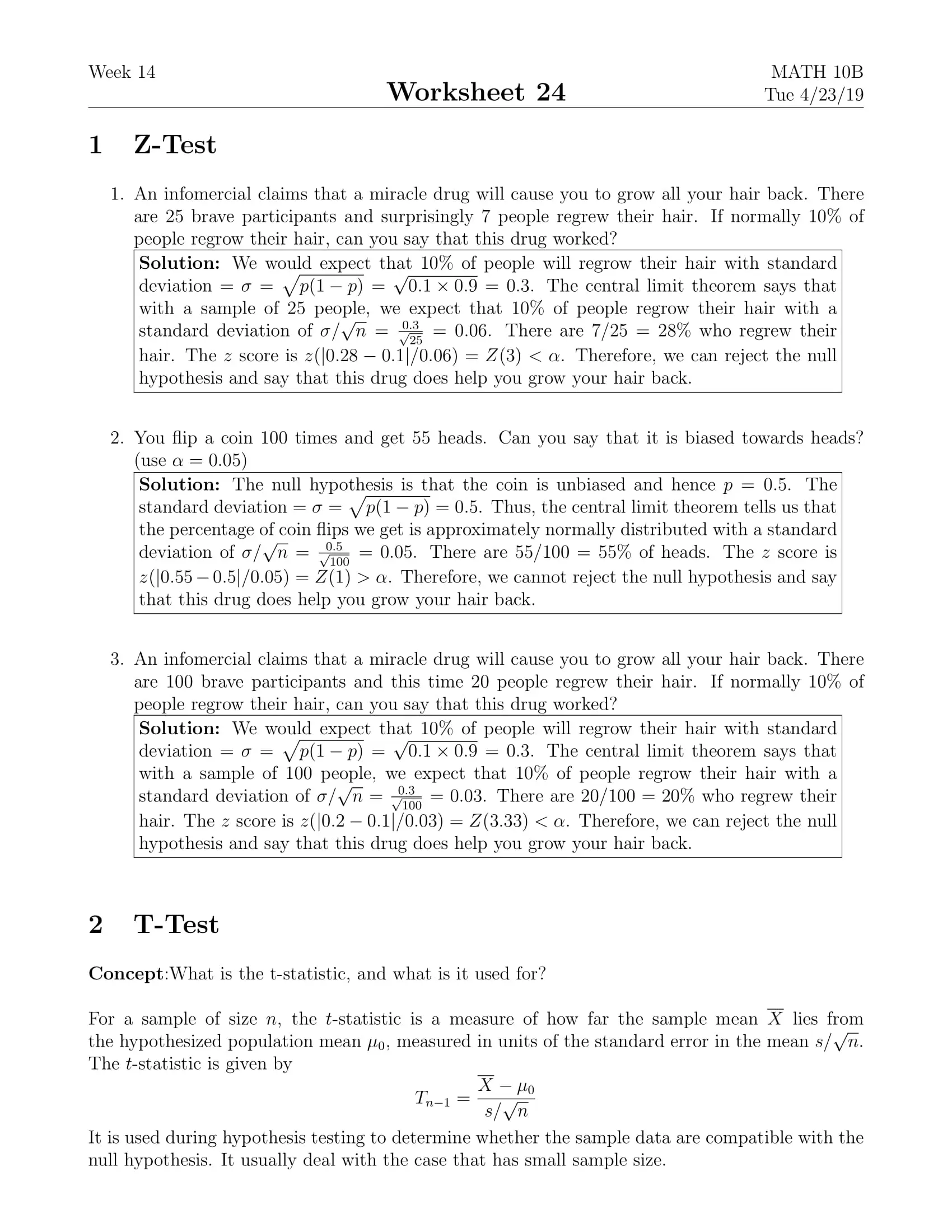Paired Sample T-Test Worksheet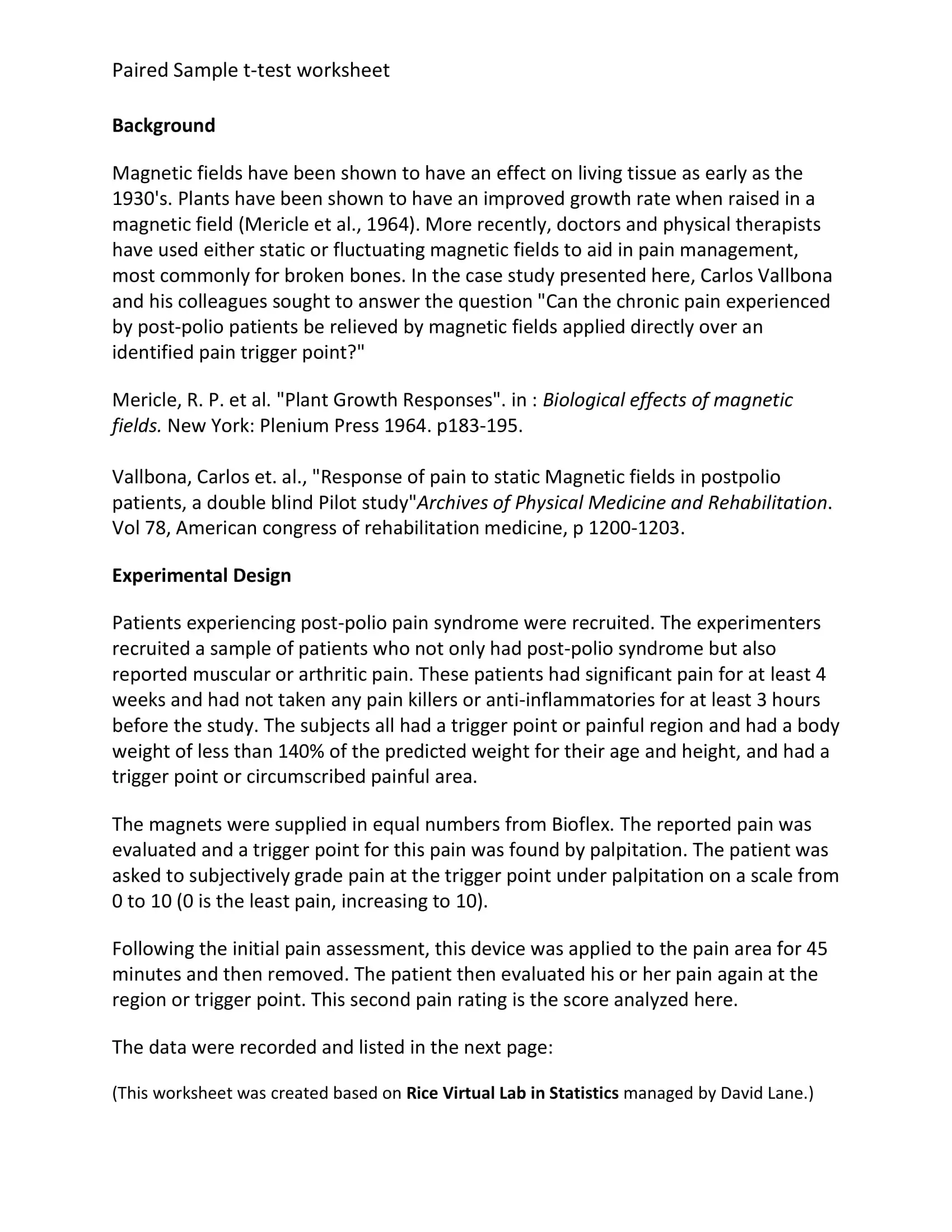Inference on a Population Mean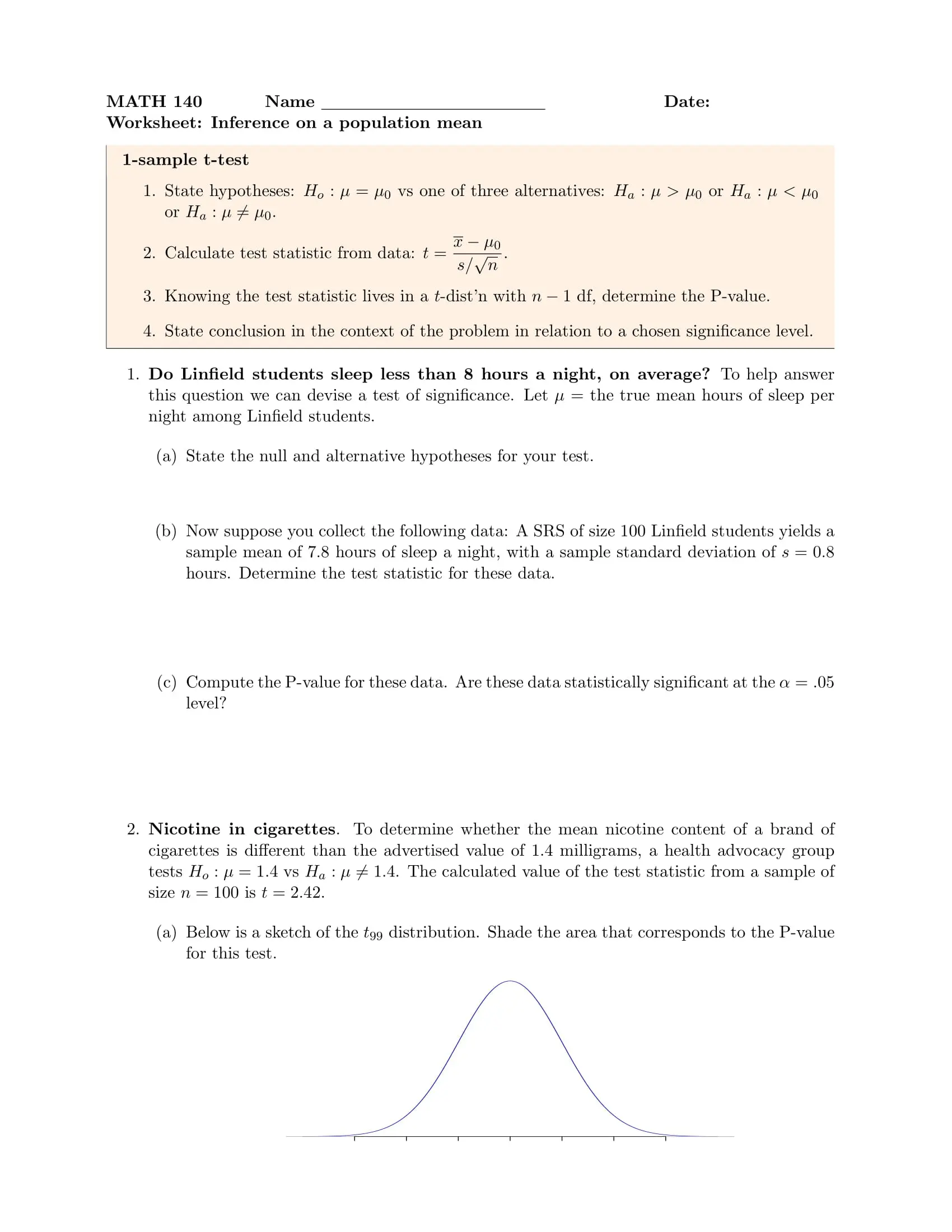T-test and Z-testThanksgiving Statistics Activity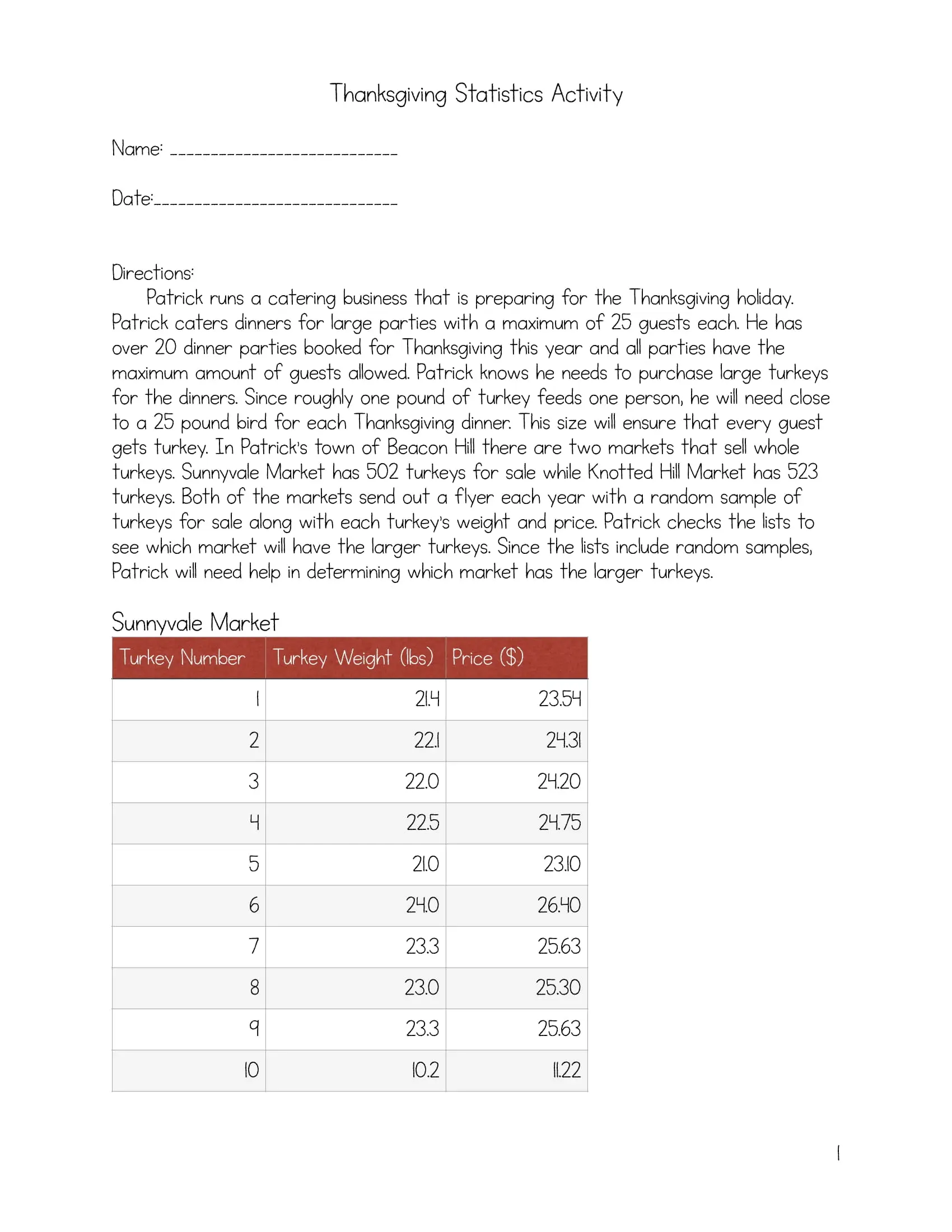Dependent T-Test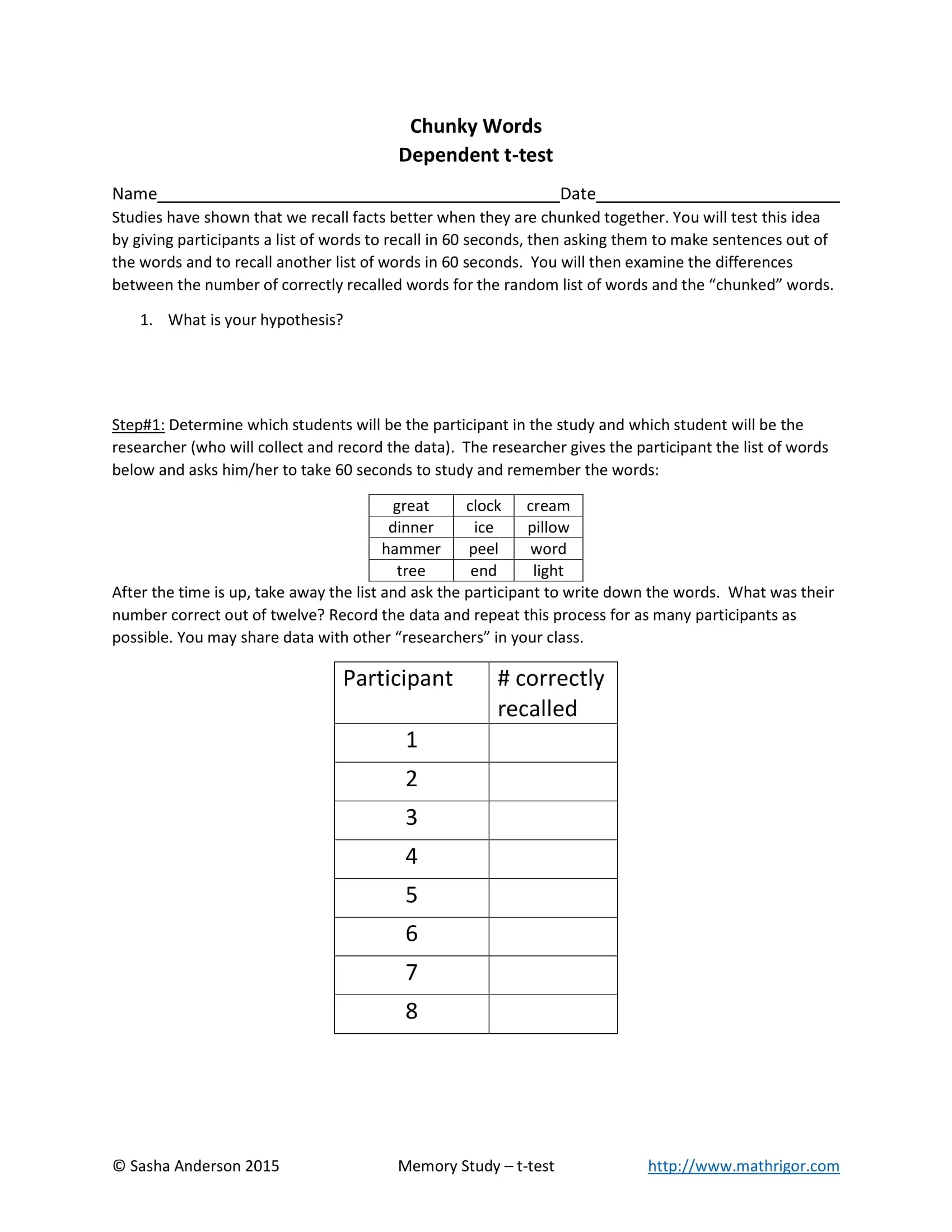Extra Problems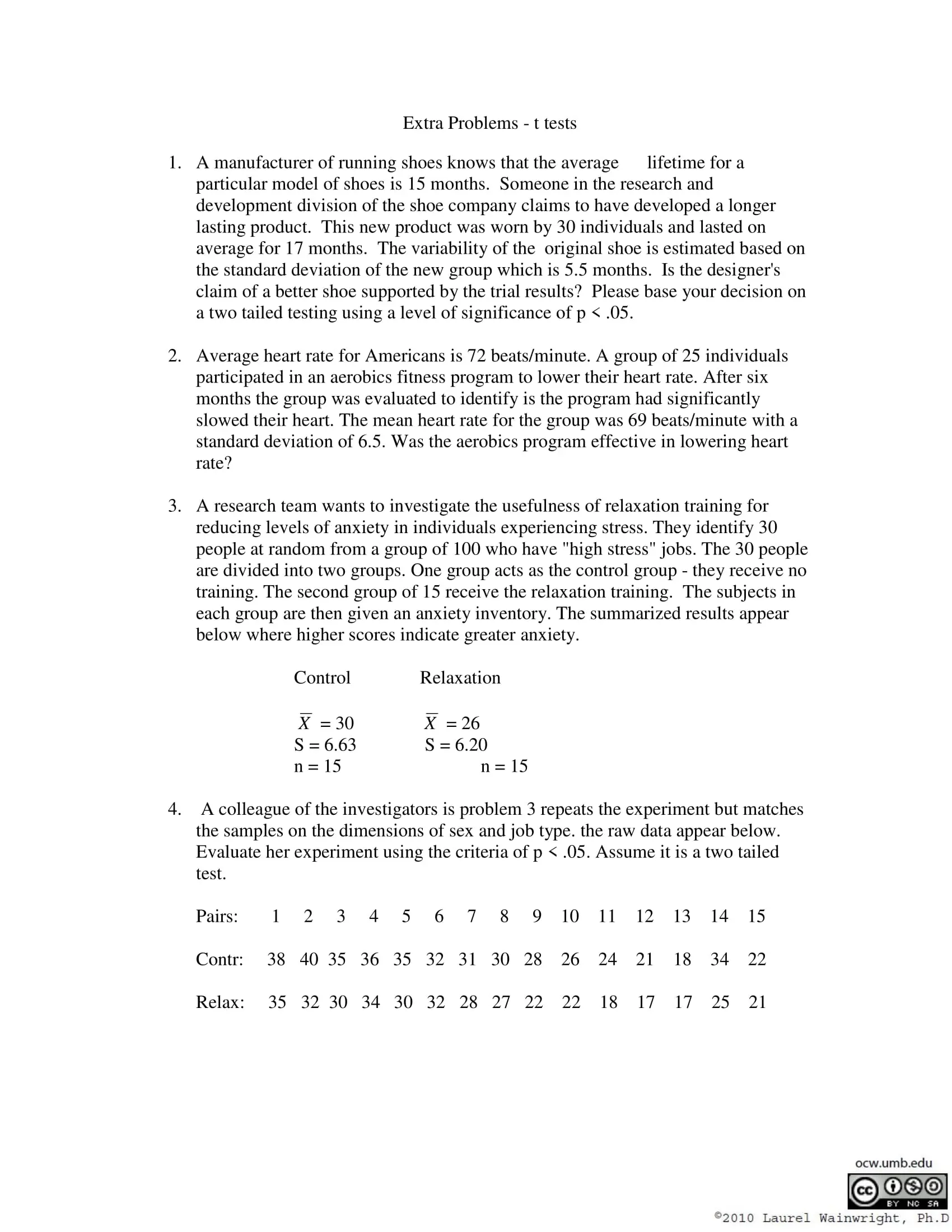Matrix Addition and Subtraction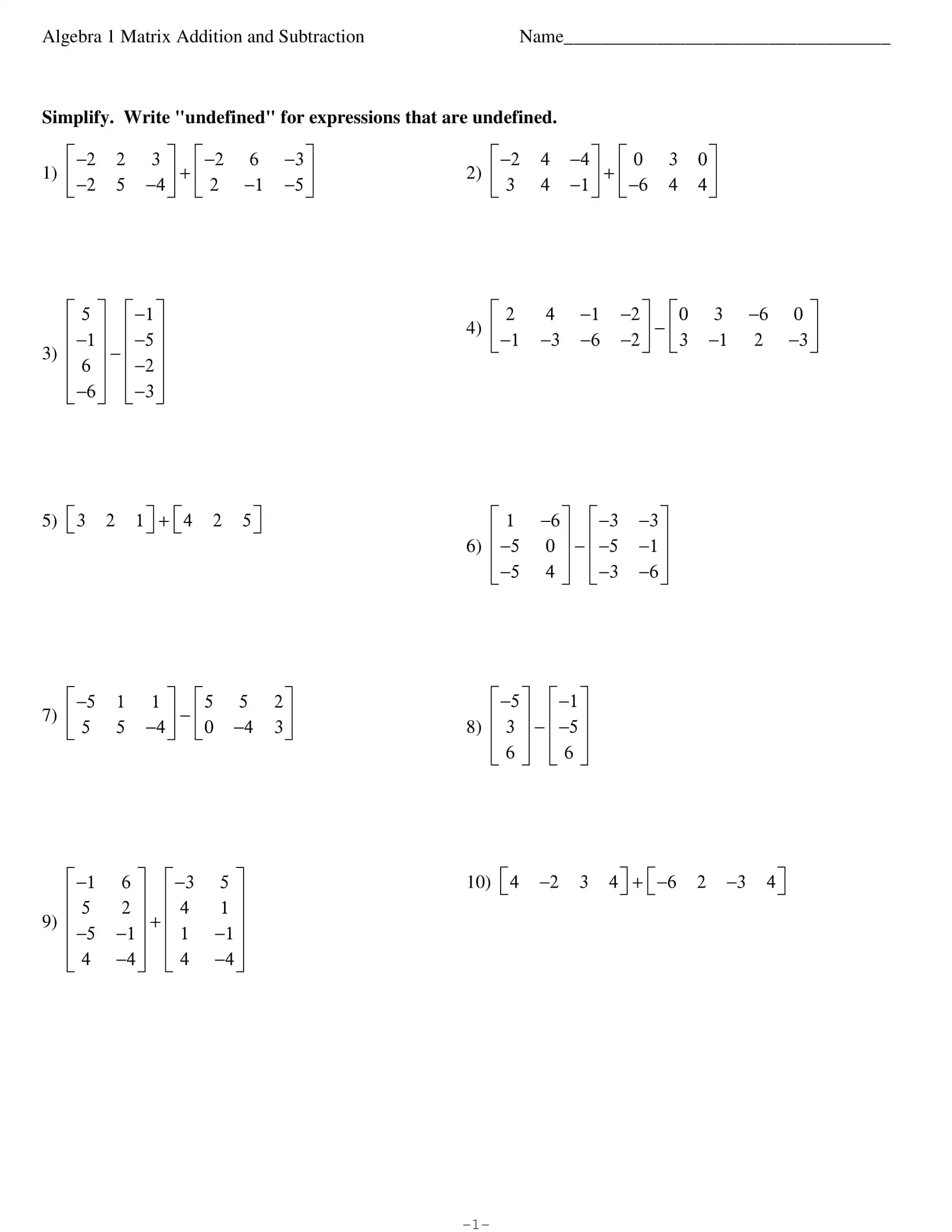All Matrix Operations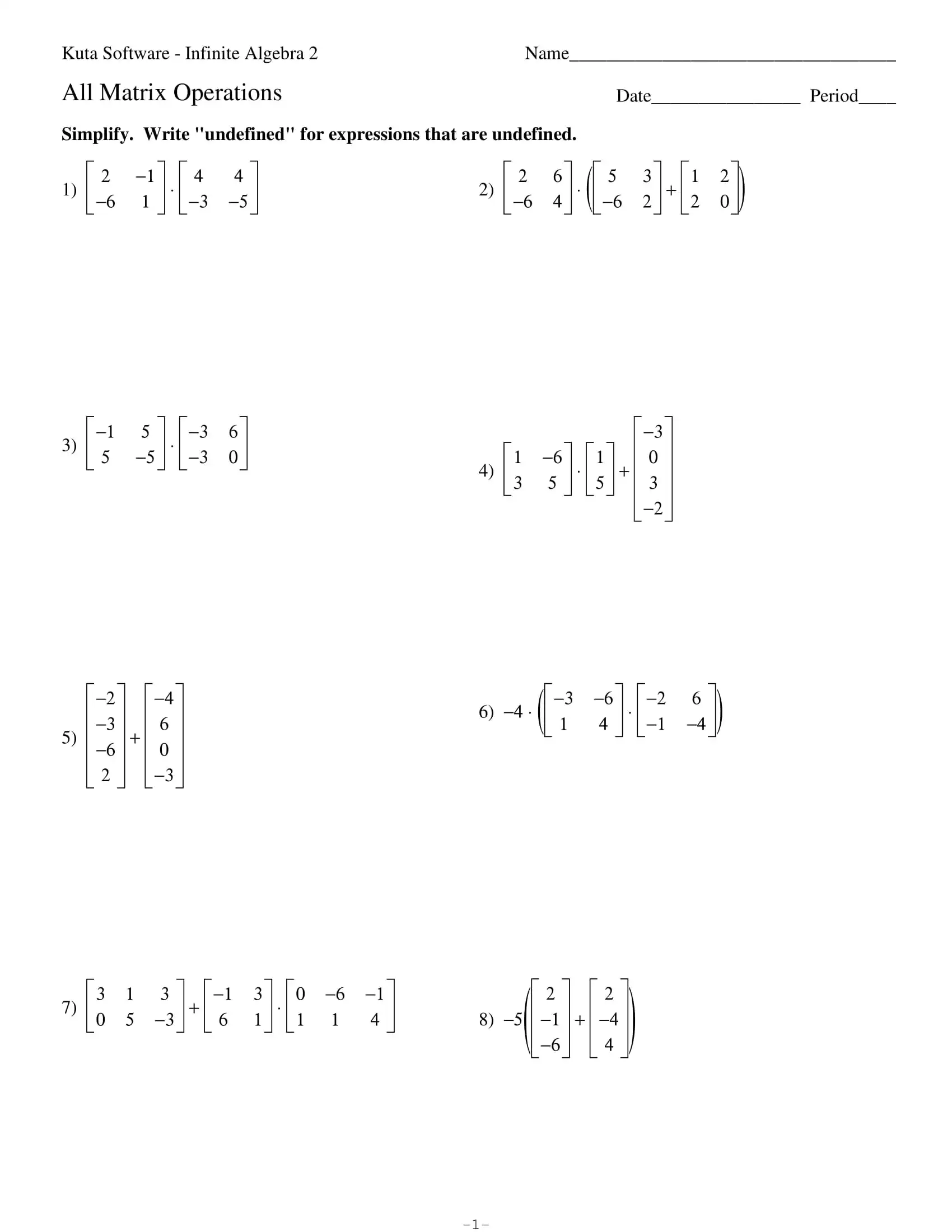Matrix Worksheet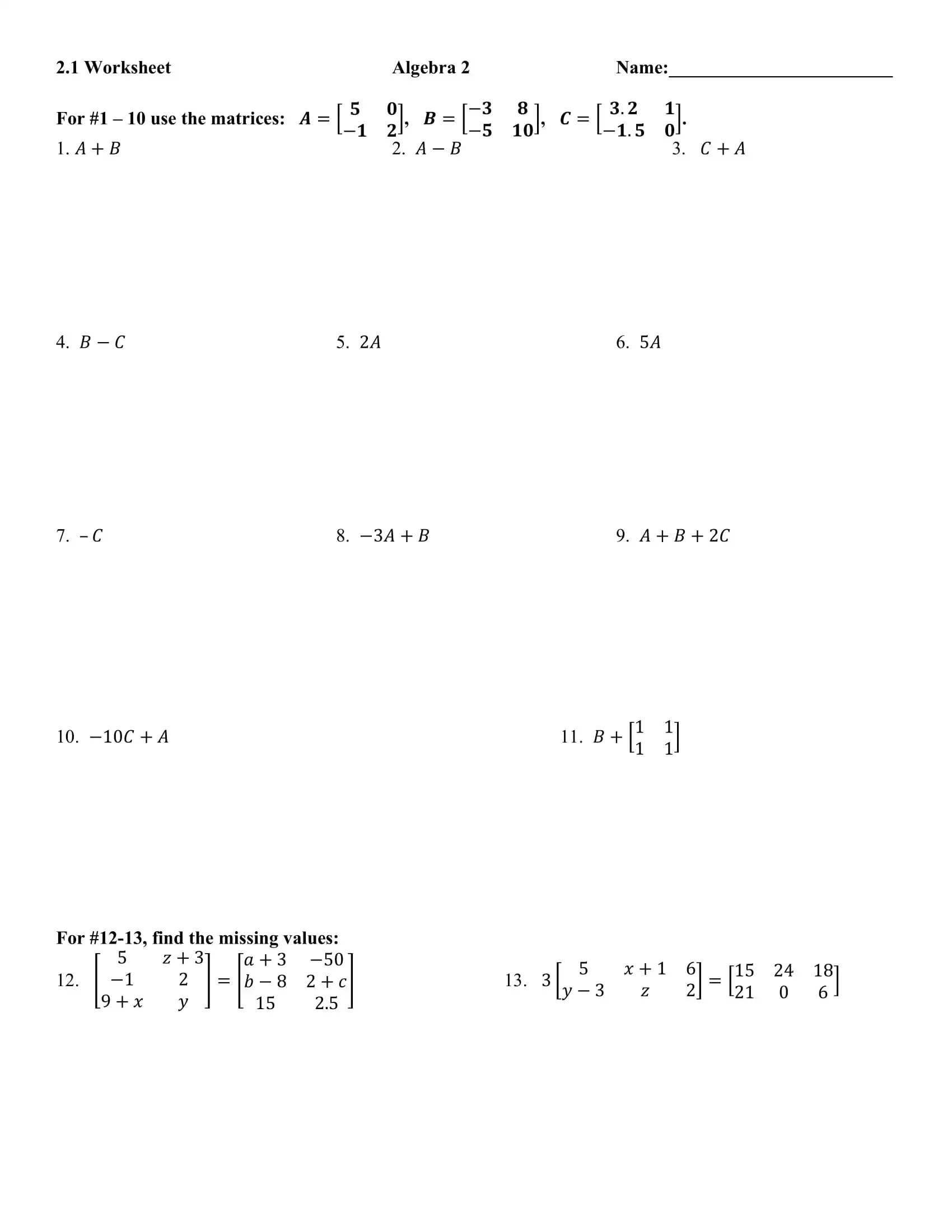Basic Matrix Operations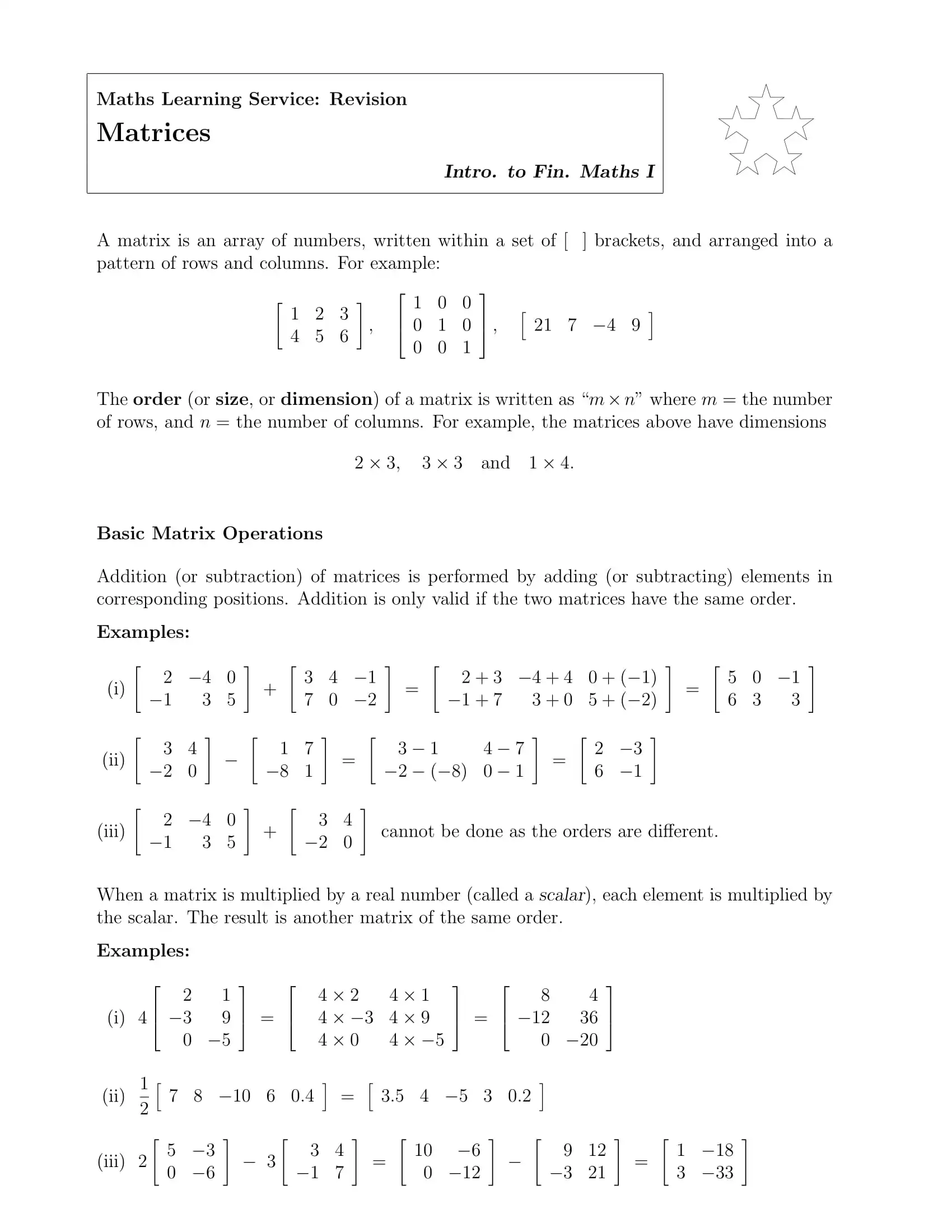Matrix Multiplication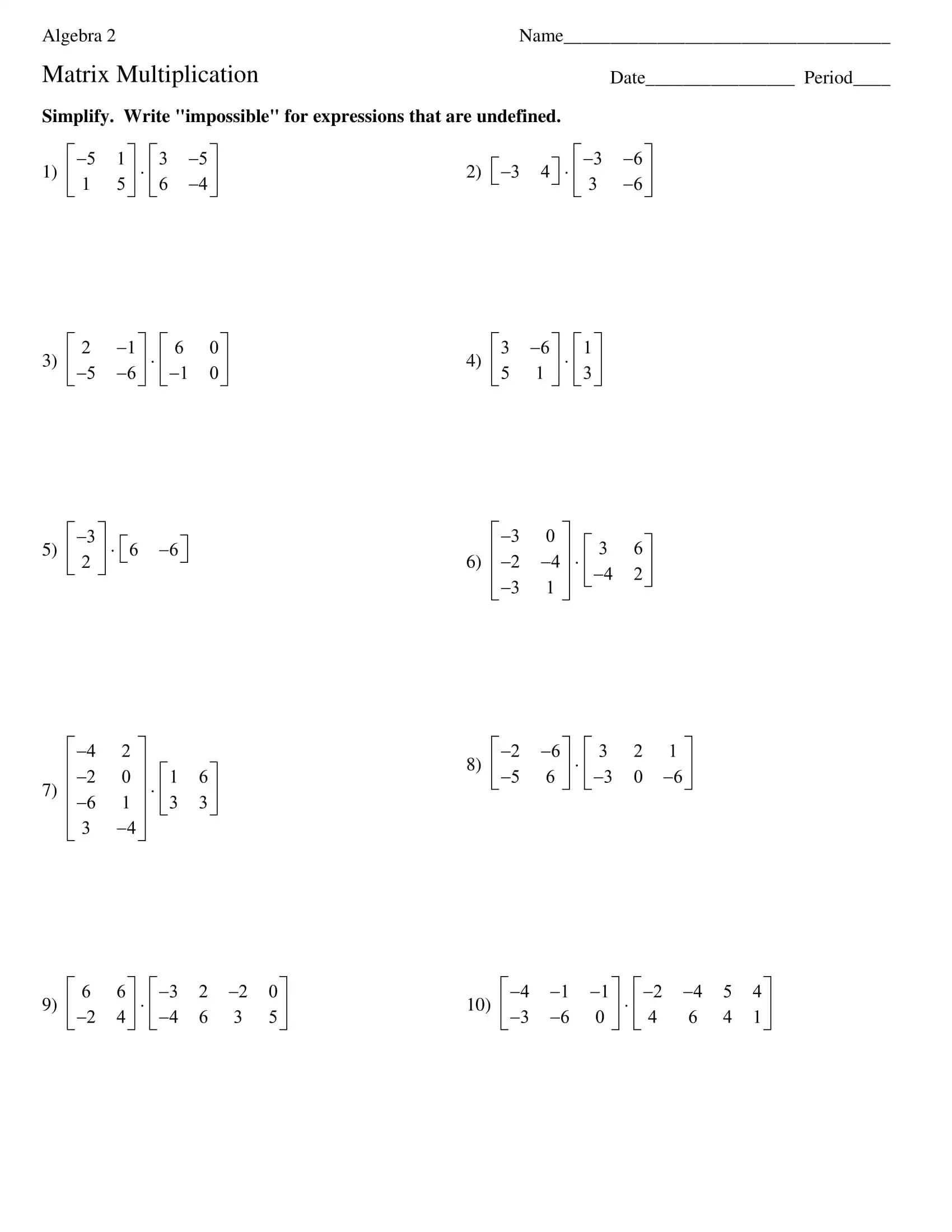Matrix Multiplication 2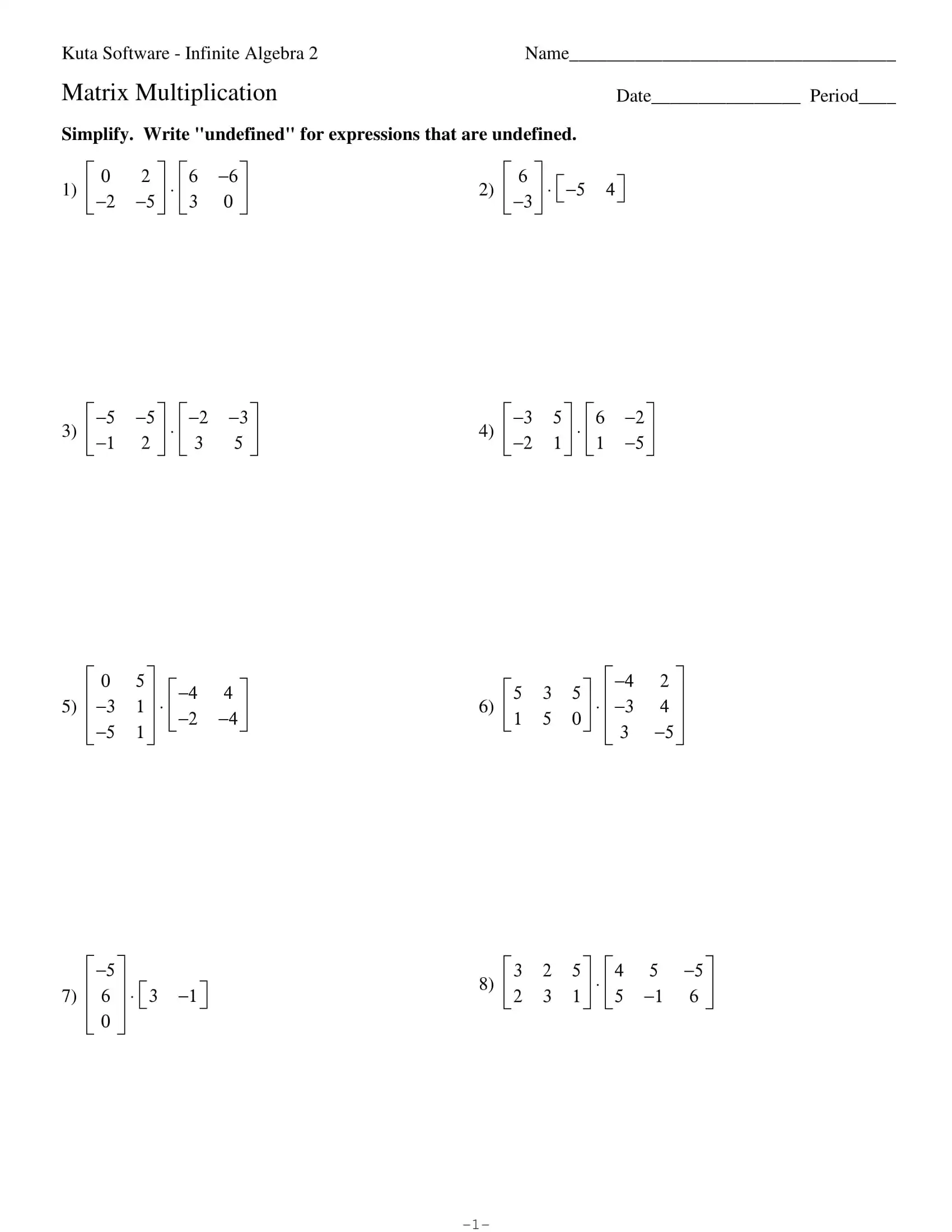Matrix Multiplication 3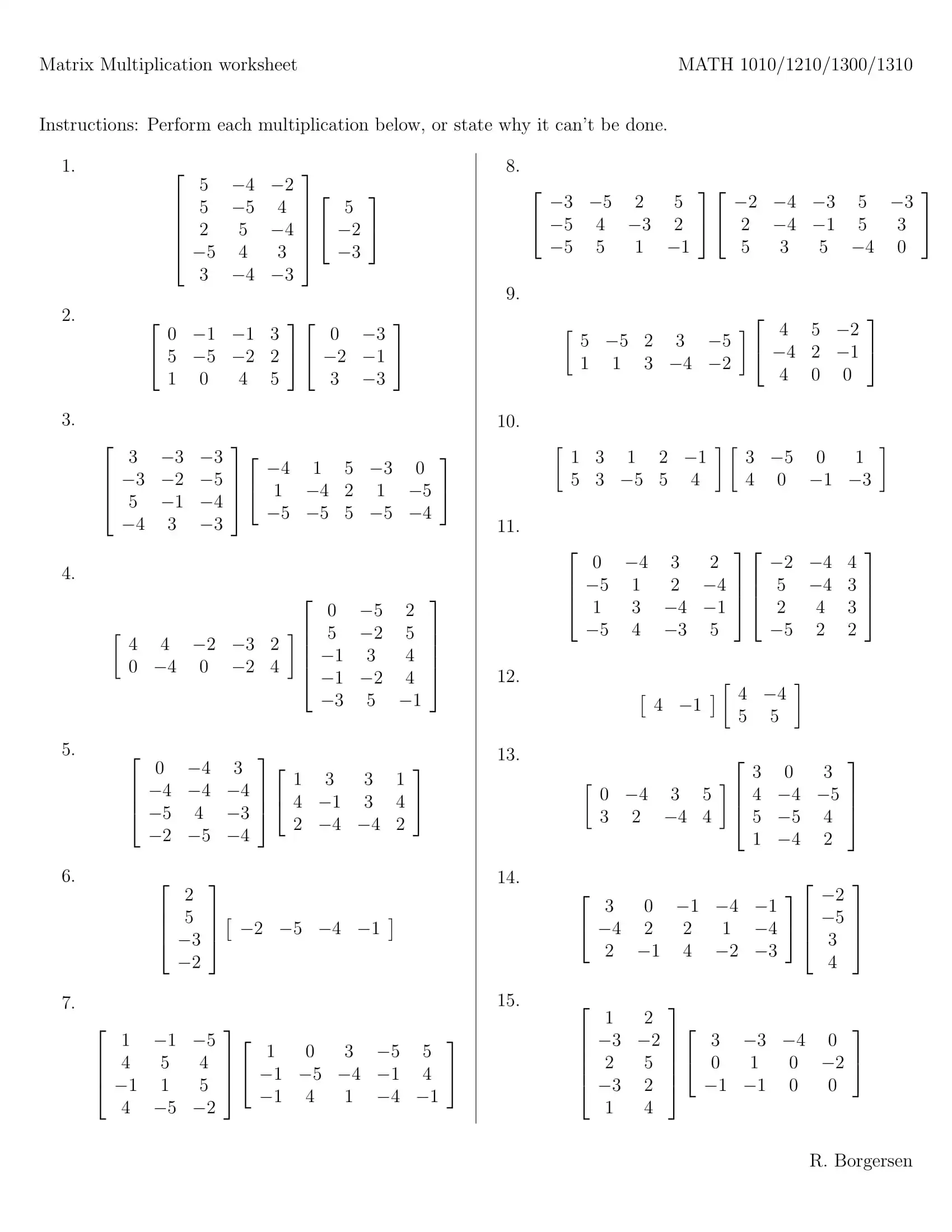Matrix Basics WorksheetFinal Grade Calculation Worksheet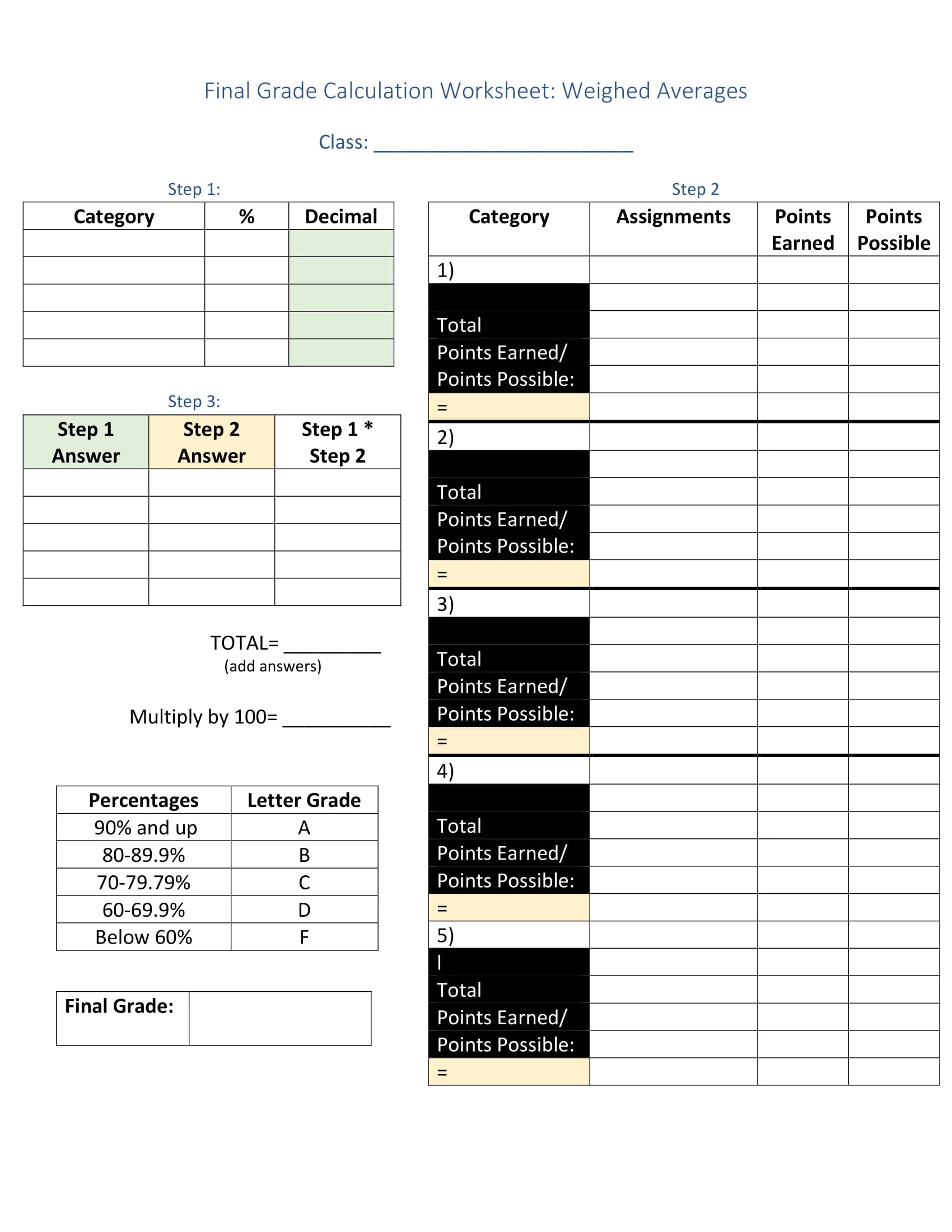Grade Calculation Worksheet 1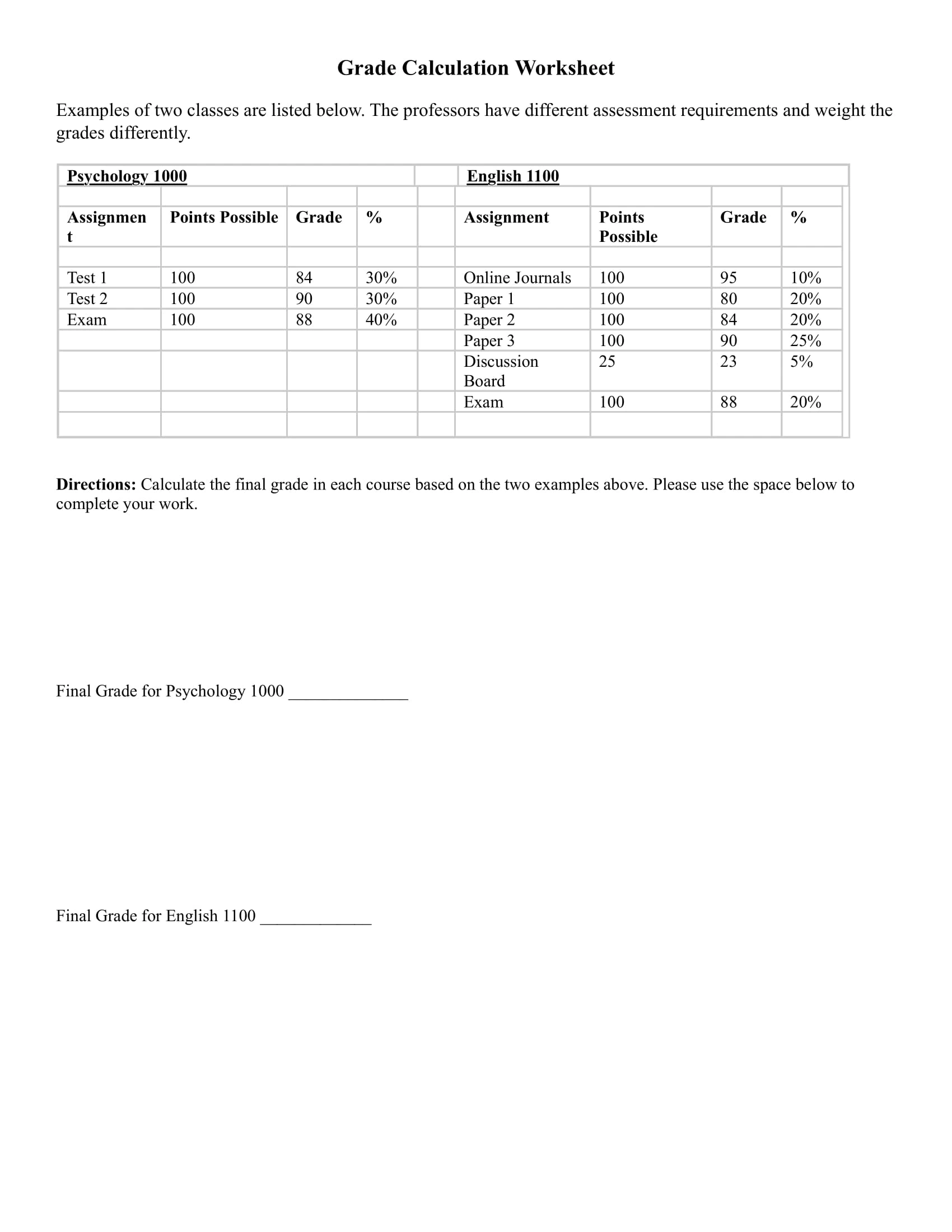Grade Calculation Worksheet 2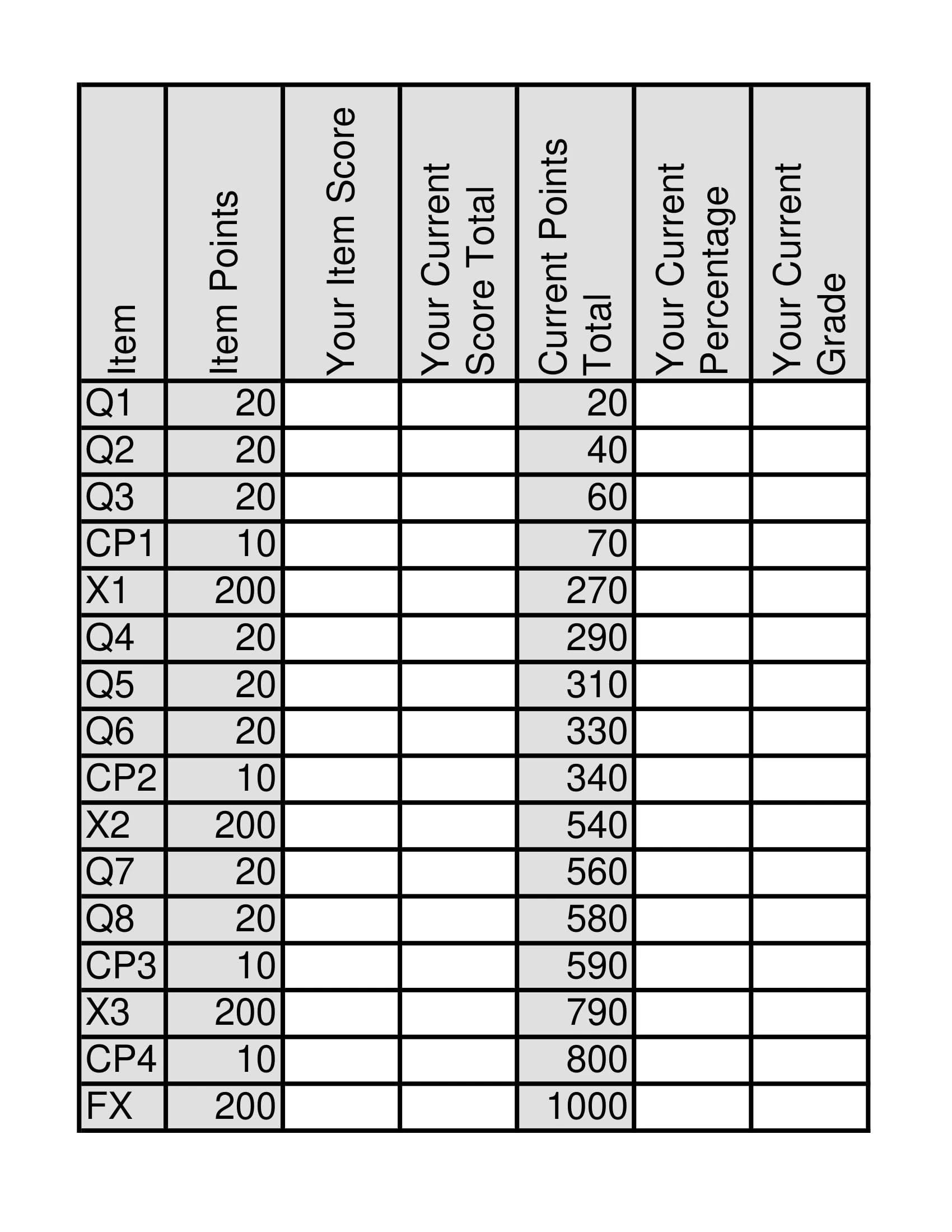Grade Calculation Worksheet 3